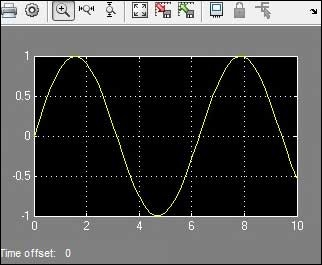# MATLAB - Quick Guide

#### Data Preprocessing for Machine Learning using MATLAB

30 Lectures 4 hours

#### Image Processing Toolbox in MATLAB

17 Lectures 3 hours

#### Matlab - The Complete Course

Best Seller

37 Lectures 5 hours

# MATLAB - Overview

MATLAB (matrix laboratory) is a fourth-generation high-level programming language and interactive environment for numerical computation, visualization and programming.

MATLAB is developed by MathWorks.

It allows matrix manipulations; plotting of functions and data; implementation of algorithms; creation of user interfaces; interfacing with programs written in other languages, including C, C++, Java, and FORTRAN; analyze data; develop algorithms; and create models and applications.

It has numerous built-in commands and math functions that help you in mathematical calculations, generating plots, and performing numerical methods.

## MATLAB's Power of Computational Mathematics

MATLAB is used in every facet of computational mathematics. Following are some commonly used mathematical calculations where it is used most commonly −

• Dealing with Matrices and Arrays
• 2-D and 3-D Plotting and graphics
• Linear Algebra
• Algebraic Equations
• Non-linear Functions
• Statistics
• Data Analysis
• Calculus and Differential Equations
• Numerical Calculations
• Integration
• Transforms
• Curve Fitting
• Various other special functions

## Features of MATLAB

Following are the basic features of MATLAB −

• It is a high-level language for numerical computation, visualization and application development.

• It also provides an interactive environment for iterative exploration, design and problem solving.

• It provides vast library of mathematical functions for linear algebra, statistics, Fourier analysis, filtering, optimization, numerical integration and solving ordinary differential equations.

• It provides built-in graphics for visualizing data and tools for creating custom plots.

• MATLAB's programming interface gives development tools for improving code quality maintainability and maximizing performance.

• It provides tools for building applications with custom graphical interfaces.

• It provides functions for integrating MATLAB based algorithms with external applications and languages such as C, Java, .NET and Microsoft Excel.

## Uses of MATLAB

MATLAB is widely used as a computational tool in science and engineering encompassing the fields of physics, chemistry, math and all engineering streams. It is used in a range of applications including −

• Signal Processing and Communications
• Image and Video Processing
• Control Systems
• Test and Measurement
• Computational Finance
• Computational Biology

# MATLAB - Environment Setup

## Local Environment Setup

Setting up MATLAB environment is a matter of few clicks. The installer can be downloaded from here.

MathWorks provides the licensed product, a trial version and a student version as well. You need to log into the site and wait a little for their approval.

After downloading the installer the software can be installed through few clicks.## Understanding the MATLAB Environment

MATLAB development IDE can be launched from the icon created on the desktop. The main working window in MATLAB is called the desktop. When MATLAB is started, the desktop appears in its default layout −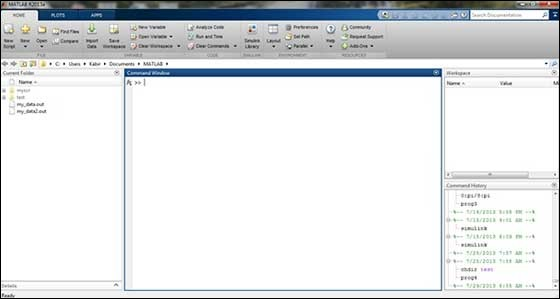The desktop has the following panels −

• Current Folder − This panel allows you to access the project folders and files.

•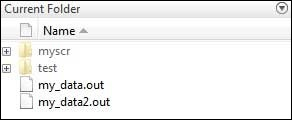• Command Window − This is the main area where commands can be entered at the command line. It is indicated by the command prompt (>>).

•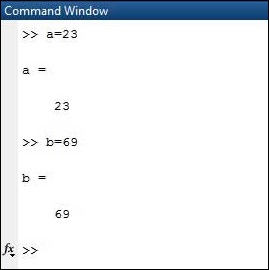• Workspace − The workspace shows all the variables created and/or imported from files.

•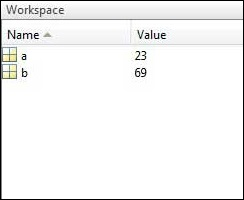• Command History − This panel shows or return commands that are entered at the command line.

•## Set up GNU Octave

If you are willing to use Octave on your machine ( Linux, BSD, OS X or Windows ), then kindly download latest version from Download GNU Octave. You can check the given installation instructions for your machine.

# MATLAB - Basic Syntax

MATLAB environment behaves like a super-complex calculator. You can enter commands at the >> command prompt.

MATLAB is an interpreted environment. In other words, you give a command and MATLAB executes it right away.

## Hands on Practice

Type a valid expression, for example,

```5 + 5
```

And press ENTER

When you click the Execute button, or type Ctrl+E, MATLAB executes it immediately and the result returned is −

```ans = 10
```

Let us take up few more examples −

```3 ^ 2	       % 3 raised to the power of 2
```

When you click the Execute button, or type Ctrl+E, MATLAB executes it immediately and the result returned is −

```ans = 9
```

Another example,

```sin(pi /2)	  % sine of angle 90o
```

When you click the Execute button, or type Ctrl+E, MATLAB executes it immediately and the result returned is −

```ans = 1
```

Another example,

```7/0		      % Divide by zero
```

When you click the Execute button, or type Ctrl+E, MATLAB executes it immediately and the result returned is −

```ans = Inf
warning: division by zero
```

Another example,

```732 * 20.3
```

When you click the Execute button, or type Ctrl+E, MATLAB executes it immediately and the result returned is −

```ans =  1.4860e+04
```

MATLAB provides some special expressions for some mathematical symbols, like pi for π, Inf for ∞, i (and j) for √-1 etc. Nan stands for 'not a number'.

## Use of Semicolon (;) in MATLAB

Semicolon (;) indicates end of statement. However, if you want to suppress and hide the MATLAB output for an expression, add a semicolon after the expression.

For example,

```x = 3;
y = x + 5
```

When you click the Execute button, or type Ctrl+E, MATLAB executes it immediately and the result returned is −

```y =  8
```

The percent symbol (%) is used for indicating a comment line. For example,

```x = 9	     % assign the value 9 to x
```

You can also write a block of comments using the block comment operators % { and % }.

The MATLAB editor includes tools and context menu items to help you add, remove, or change the format of comments.

## Commonly used Operators and Special Characters

MATLAB supports the following commonly used operators and special characters −

Operator Purpose
+ Plus; addition operator.
- Minus; subtraction operator.
* Scalar and matrix multiplication operator.
.* Array multiplication operator.
^ Scalar and matrix exponentiation operator.
.^ Array exponentiation operator.
\ Left-division operator.
/ Right-division operator.
.\ Array left-division operator.
./ Array right-division operator.
: Colon; generates regularly spaced elements and represents an entire row or column.
( ) Parentheses; encloses function arguments and array indices; overrides precedence.
[ ] Brackets; enclosures array elements.
. Decimal point.
Ellipsis; line-continuation operator
, Comma; separates statements and elements in a row
; Semicolon; separates columns and suppresses display.
% Percent sign; designates a comment and specifies formatting.
_ Quote sign and transpose operator.
._ Nonconjugated transpose operator.
= Assignment operator.

## Special Variables and Constants

MATLAB supports the following special variables and constants −

Name Meaning
ans Most recent answer.
eps Accuracy of floating-point precision.
i,j The imaginary unit √-1.
Inf Infinity.
NaN Undefined numerical result (not a number).
pi The number π

## Naming Variables

Variable names consist of a letter followed by any number of letters, digits or underscore.

MATLAB is case-sensitive.

Variable names can be of any length, however, MATLAB uses only first N characters, where N is given by the function namelengthmax.

## Saving Your Work

The save command is used for saving all the variables in the workspace, as a file with .mat extension, in the current directory.

For example,

```save myfile
```

You can reload the file anytime later using the load command.

```load myfile
```

# MATLAB - Variables

In MATLAB environment, every variable is an array or matrix.

You can assign variables in a simple way. For example,

```x = 3	       % defining x and initializing it with a value
```

MATLAB will execute the above statement and return the following result −

```x = 3
```

It creates a 1-by-1 matrix named x and stores the value 3 in its element. Let us check another example,

```x = sqrt(16) 	% defining x and initializing it with an expression
```

MATLAB will execute the above statement and return the following result −

```x = 4
```

Please note that −

• Once a variable is entered into the system, you can refer to it later.

• Variables must have values before they are used.

• When an expression returns a result that is not assigned to any variable, the system assigns it to a variable named ans, which can be used later.

For example,

```sqrt(78)
```

MATLAB will execute the above statement and return the following result −

```ans =  8.8318
```

You can use this variable ans

```sqrt(78);
9876/ans
```

MATLAB will execute the above statement and return the following result −

```ans =  1118.2
```

Let's look at another example −

```x = 7 * 8;
y = x * 7.89
```

MATLAB will execute the above statement and return the following result −

```y =  441.84
```

## Multiple Assignments

You can have multiple assignments on the same line. For example,

```a = 2; b = 7; c = a * b
```

MATLAB will execute the above statement and return the following result −

```c = 14
```

## I have forgotten the Variables!

The who command displays all the variable names you have used.

```who
```

MATLAB will execute the above statement and return the following result −

```Your variables are:
a    ans  b    c
```

The whos command displays little more about the variables −

• Variables currently in memory
• Type of each variables
• Memory allocated to each variable
• Whether they are complex variables or not
```whos
```

MATLAB will execute the above statement and return the following result −

```Attr Name        Size        Bytes      Class
==== ====        ====        ====       =====
a             1x1           8        double
ans           1x70         757        cell
b             1x1           8        double
c             1x1           8        double

Total is 73 elements using 781 bytes
```

The clear command deletes all (or the specified) variable(s) from the memory.

```clear x     % it will delete x, won't display anything
clear       % it will delete all variables in the workspace
%  peacefully and unobtrusively
```

## Long Assignments

Long assignments can be extended to another line by using an ellipses (...). For example,

```initial_velocity = 0;
acceleration = 9.8;
time = 20;
final_velocity = initial_velocity + acceleration * time
```

MATLAB will execute the above statement and return the following result −

```final_velocity = 196
```

## The format Command

By default, MATLAB displays numbers with four decimal place values. This is known as short format.

However, if you want more precision, you need to use the format command.

The format long command displays 16 digits after decimal.

For example −

```format long
x = 7 + 10/3 + 5 ^ 1.2
```

MATLAB will execute the above statement and return the following result−

```x = 17.2319816406394
```

Another example,

```format short
x = 7 + 10/3 + 5 ^ 1.2
```

MATLAB will execute the above statement and return the following result −

```x = 17.232
```

The format bank command rounds numbers to two decimal places. For example,

```format bank
daily_wage = 177.45;
weekly_wage = daily_wage * 6
```

MATLAB will execute the above statement and return the following result −

```weekly_wage = 1064.70
```

MATLAB displays large numbers using exponential notation.

The format short e command allows displaying in exponential form with four decimal places plus the exponent.

For example,

```format short e
4.678 * 4.9
```

MATLAB will execute the above statement and return the following result −

```ans = 2.2922e+01
```

The format long e command allows displaying in exponential form with four decimal places plus the exponent. For example,

```format long e
x = pi
```

MATLAB will execute the above statement and return the following result −

```x = 3.141592653589793e+00
```

The format rat command gives the closest rational expression resulting from a calculation. For example,

```format rat
4.678 * 4.9
```

MATLAB will execute the above statement and return the following result −

```ans = 34177/1491
```

## Creating Vectors

A vector is a one-dimensional array of numbers. MATLAB allows creating two types of vectors −

• Row vectors
• Column vectors

Row vectors are created by enclosing the set of elements in square brackets, using space or comma to delimit the elements.

For example,

```r = [7 8 9 10 11]
```

MATLAB will execute the above statement and return the following result −

```r =

7    8    9   10   11
```

Another example,

```r = [7 8 9 10 11];
t = [2, 3, 4, 5, 6];
res = r + t
```

MATLAB will execute the above statement and return the following result −

```res =

9         11         13         15         17
```

Column vectors are created by enclosing the set of elements in square brackets, using semicolon(;) to delimit the elements.

```c = [7;  8;  9;  10; 11]
```

MATLAB will execute the above statement and return the following result −

```c =
7
8
9
10
11
```

## Creating Matrices

A matrix is a two-dimensional array of numbers.

In MATLAB, a matrix is created by entering each row as a sequence of space or comma separated elements, and end of a row is demarcated by a semicolon. For example, let us create a 3-by-3 matrix as −

```m = [1 2 3; 4 5 6; 7 8 9]
```

MATLAB will execute the above statement and return the following result −

```m =
1              2              3
4              5              6
7              8              9
```

# MATLAB - Commands

MATLAB is an interactive program for numerical computation and data visualization. You can enter a command by typing it at the MATLAB prompt '>>' on the Command Window.

In this section, we will provide lists of commonly used general MATLAB commands.

## Commands for Managing a Session

MATLAB provides various commands for managing a session. The following table provides all such commands −

Command Purpose
clc Clears command window.
clear Removes variables from memory.
exist Checks for existence of file or variable.
global Declares variables to be global.
help Searches for a help topic.
lookfor Searches help entries for a keyword.
quit Stops MATLAB.
who Lists current variables.
whos Lists current variables (long display).

## Commands for Working with the System

MATLAB provides various useful commands for working with the system, like saving the current work in the workspace as a file and loading the file later.

It also provides various commands for other system-related activities like, displaying date, listing files in the directory, displaying current directory, etc.

The following table displays some commonly used system-related commands −

Command Purpose
cd Changes current directory.
date Displays current date.
delete Deletes a file.
diary Switches on/off diary file recording.
dir Lists all files in current directory.
load Loads workspace variables from a file.
path Displays search path.
pwd Displays current directory.
save Saves workspace variables in a file.
type Displays contents of a file.
what Lists all MATLAB files in the current directory.

## Input and Output Commands

MATLAB provides the following input and output related commands −

Command Purpose
disp Displays contents of an array or string.
fscanf Read formatted data from a file.
format Controls screen-display format.
fprintf Performs formatted writes to screen or file.
input Displays prompts and waits for input.
; Suppresses screen printing.

The fscanf and fprintf commands behave like C scanf and printf functions. They support the following format codes −

Format Code Purpose
%s Format as a string.
%d Format as an integer.
%f Format as a floating point value.
%e Format as a floating point value in scientific notation.
%g Format in the most compact form: %f or %e.
\n Insert a new line in the output string.
\t Insert a tab in the output string.

The format function has the following forms used for numeric display −

Format Function Display up to
format short Four decimal digits (default).
format long 16 decimal digits.
format short e Five digits plus exponent.
format long e 16 digits plus exponents.
format bank Two decimal digits.
format + Positive, negative, or zero.
format rat Rational approximation.
format compact Suppresses some line feeds.
format loose Resets to less compact display mode.

## Vector, Matrix and Array Commands

The following table shows various commands used for working with arrays, matrices and vectors −

Command Purpose
cat Concatenates arrays.
find Finds indices of nonzero elements.
length Computes number of elements.
linspace Creates regularly spaced vector.
logspace Creates logarithmically spaced vector.
max Returns largest element.
min Returns smallest element.
prod Product of each column.
reshape Changes size.
size Computes array size.
sort Sorts each column.
sum Sums each column.
eye Creates an identity matrix.
ones Creates an array of ones.
zeros Creates an array of zeros.
cross Computes matrix cross products.
dot Computes matrix dot products.
det Computes determinant of an array.
inv Computes inverse of a matrix.
pinv Computes pseudoinverse of a matrix.
rank Computes rank of a matrix.
rref Computes reduced row echelon form.
cell Creates cell array.
celldisp Displays cell array.
cellplot Displays graphical representation of cell array.
num2cell Converts numeric array to cell array.
deal Matches input and output lists.
iscell Identifies cell array.

## Plotting Commands

MATLAB provides numerous commands for plotting graphs. The following table shows some of the commonly used commands for plotting −

Command Purpose
axis Sets axis limits.
fplot Intelligent plotting of functions.
grid Displays gridlines.
plot Generates xy plot.
print Prints plot or saves plot to a file.
title Puts text at top of plot.
xlabel Adds text label to x-axis.
ylabel Adds text label to y-axis.
axes Creates axes objects.
close Closes the current plot.
close all Closes all plots.
figure Opens a new figure window.
gtext Enables label placement by mouse.
hold Freezes current plot.
legend Legend placement by mouse.
refresh Redraws current figure window.
set Specifies properties of objects such as axes.
subplot Creates plots in subwindows.
text Places string in figure.
bar Creates bar chart.
loglog Creates log-log plot.
polar Creates polar plot.
semilogx Creates semilog plot. (logarithmic abscissa).
semilogy Creates semilog plot. (logarithmic ordinate).
stairs Creates stairs plot.
stem Creates stem plot.

# MATLAB - M-Files

So far, we have used MATLAB environment as a calculator. However, MATLAB is also a powerful programming language, as well as an interactive computational environment.

In previous chapters, you have learned how to enter commands from the MATLAB command prompt. MATLAB also allows you to write series of commands into a file and execute the file as complete unit, like writing a function and calling it.

## The M Files

MATLAB allows writing two kinds of program files −

• Scripts − script files are program files with .m extension. In these files, you write series of commands, which you want to execute together. Scripts do not accept inputs and do not return any outputs. They operate on data in the workspace.

• Functions − functions files are also program files with .m extension. Functions can accept inputs and return outputs. Internal variables are local to the function.

You can use the MATLAB editor or any other text editor to create your .mfiles. In this section, we will discuss the script files. A script file contains multiple sequential lines of MATLAB commands and function calls. You can run a script by typing its name at the command line.

## Creating and Running Script File

To create scripts files, you need to use a text editor. You can open the MATLAB editor in two ways −

• Using the command prompt
• Using the IDE

If you are using the command prompt, type edit in the command prompt. This will open the editor. You can directly type edit and then the filename (with .m extension)

```edit
Or
edit <filename>
```

The above command will create the file in default MATLAB directory. If you want to store all program files in a specific folder, then you will have to provide the entire path.

Let us create a folder named progs. Type the following commands at the command prompt (>>) −

```mkdir progs    % create directory progs under default directory
chdir progs    % changing the current directory to progs
edit  prog1.m  % creating an m file named prog1.m
```

If you are creating the file for first time, MATLAB prompts you to confirm it. Click Yes.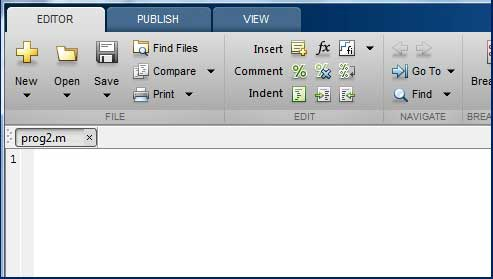Alternatively, if you are using the IDE, choose NEW -> Script. This also opens the editor and creates a file named Untitled. You can name and save the file after typing the code.

Type the following code in the editor −

```NoOfStudents = 6000;
TeachingStaff = 150;
NonTeachingStaff = 20;
Total = NoOfStudents + TeachingStaff ...
+ NonTeachingStaff;
disp(Total);
```

After creating and saving the file, you can run it in two ways −

• Clicking the Run button on the editor window or

• Just typing the filename (without extension) in the command prompt: >> prog1

The command window prompt displays the result −

```6170
```

### Example

Create a script file, and type the following code −

```a = 5; b = 7;
c = a + b
d = c + sin(b)
e = 5 * d
f = exp(-d)
```

When the above code is compiled and executed, it produces the following result −

```c =  12
d =  12.657
e =  63.285
f =    3.1852e-06
```

# MATLAB - Data Types

MATLAB does not require any type declaration or dimension statements. Whenever MATLAB encounters a new variable name, it creates the variable and allocates appropriate memory space.

If the variable already exists, then MATLAB replaces the original content with new content and allocates new storage space, where necessary.

For example,

```Total = 42
```

The above statement creates a 1-by-1 matrix named 'Total' and stores the value 42 in it.

## Data Types Available in MATLAB

MATLAB provides 15 fundamental data types. Every data type stores data that is in the form of a matrix or array. The size of this matrix or array is a minimum of 0-by-0 and this can grow up to a matrix or array of any size.

The following table shows the most commonly used data types in MATLAB −

Sr.No. Data Type & Description
1

int8

8-bit signed integer

2

uint8

8-bit unsigned integer

3

int16

16-bit signed integer

4

uint16

16-bit unsigned integer

5

int32

32-bit signed integer

6

uint32

32-bit unsigned integer

7

int64

64-bit signed integer

8

uint64

64-bit unsigned integer

9

single

single precision numerical data

10

double

double precision numerical data

11

logical

logical values of 1 or 0, represent true and false respectively

12

char

character data (strings are stored as vector of characters)

13

cell array

array of indexed cells, each capable of storing an array of a different dimension and data type

14

structure

C-like structures, each structure having named fields capable of storing an array of a different dimension and data type

15

function handle

pointer to a function

16

user classes

objects constructed from a user-defined class

17

java classes

objects constructed from a Java class

### Example

Create a script file with the following code −

```str = 'Hello World!'
n = 2345
d = double(n)
un = uint32(789.50)
rn = 5678.92347
c = int32(rn)
```

When the above code is compiled and executed, it produces the following result −

```str = Hello World!
n =  2345
d =  2345
un = 790
rn = 5678.9
c =  5679
```

## Data Type Conversion

MATLAB provides various functions for converting, a value from one data type to another. The following table shows the data type conversion functions −

Function Purpose
char Convert to character array (string)
int2str Convert integer data to string
mat2str Convert matrix to string
num2str Convert number to string
str2double Convert string to double-precision value
str2num Convert string to number
native2unicode Convert numeric bytes to Unicode characters
unicode2native Convert Unicode characters to numeric bytes
base2dec Convert base N number string to decimal number
bin2dec Convert binary number string to decimal number
dec2base Convert decimal to base N number in string
dec2bin Convert decimal to binary number in string
dec2hex Convert decimal to hexadecimal number in string
hex2dec Convert hexadecimal number string to decimal number
hex2num Convert hexadecimal number string to double-precision number
num2hex Convert singles and doubles to IEEE hexadecimal strings
cell2mat Convert cell array to numeric array
cell2struct Convert cell array to structure array
cellstr Create cell array of strings from character array
mat2cell Convert array to cell array with potentially different sized cells
num2cell Convert array to cell array with consistently sized cells
struct2cell Convert structure to cell array

## Determination of Data Types

MATLAB provides various functions for identifying data type of a variable.

Following table provides the functions for determining the data type of a variable −

Function Purpose
is Detect state
isa Determine if input is object of specified class
iscell Determine whether input is cell array
iscellstr Determine whether input is cell array of strings
ischar Determine whether item is character array
isfield Determine whether input is structure array field
isfloat Determine if input is floating-point array
ishghandle True for Handle Graphics object handles
isinteger Determine if input is integer array
isjava Determine if input is Java object
islogical Determine if input is logical array
isnumeric Determine if input is numeric array
isobject Determine if input is MATLAB object
isreal Check if input is real array
isscalar Determine whether input is scalar
isstr Determine whether input is character array
isstruct Determine whether input is structure array
isvector Determine whether input is vector
class Determine class of object
validateattributes Check validity of array
whos List variables in workspace, with sizes and types

### Example

Create a script file with the following code −

```x = 3
isinteger(x)
isfloat(x)
isvector(x)
isscalar(x)
isnumeric(x)

x = 23.54
isinteger(x)
isfloat(x)
isvector(x)
isscalar(x)
isnumeric(x)

x = [1 2 3]
isinteger(x)
isfloat(x)
isvector(x)
isscalar(x)

x = 'Hello'
isinteger(x)
isfloat(x)
isvector(x)
isscalar(x)
isnumeric(x)
```

When you run the file, it produces the following result −

```x = 3
ans = 0
ans = 1
ans = 1
ans = 1
ans = 1
x = 23.540
ans = 0
ans = 1
ans = 1
ans = 1
ans = 1
x =

1          2          3

ans = 0
ans = 1
ans = 1
ans = 0
x = Hello
ans = 0
ans = 0
ans = 1
ans = 0
ans = 0
```

# MATLAB - Operators

An operator is a symbol that tells the compiler to perform specific mathematical or logical manipulations. MATLAB is designed to operate primarily on whole matrices and arrays. Therefore, operators in MATLAB work both on scalar and non-scalar data. MATLAB allows the following types of elementary operations −

• Arithmetic Operators
• Relational Operators
• Logical Operators
• Bitwise Operations
• Set Operations

## Arithmetic Operators

MATLAB allows two different types of arithmetic operations −

• Matrix arithmetic operations
• Array arithmetic operations

Matrix arithmetic operations are same as defined in linear algebra. Array operations are executed element by element, both on one-dimensional and multidimensional array.

The matrix operators and array operators are differentiated by the period (.) symbol. However, as the addition and subtraction operation is same for matrices and arrays, the operator is same for both cases. The following table gives brief description of the operators −

Show Examples

Sr.No. Operator & Description
1

+

Addition or unary plus. A+B adds the values stored in variables A and B. A and B must have the same size, unless one is a scalar. A scalar can be added to a matrix of any size.

2

-

Subtraction or unary minus. A-B subtracts the value of B from A. A and B must have the same size, unless one is a scalar. A scalar can be subtracted from a matrix of any size.

3

*

Matrix multiplication. C = A*B is the linear algebraic product of the matrices A and B. More precisely,For non-scalar A and B, the number of columns of A must be equal to the number of rows of B. A scalar can multiply a matrix of any size.

4

.*

Array multiplication. A.*B is the element-by-element product of the arrays A and B. A and B must have the same size, unless one of them is a scalar.

5

/

Slash or matrix right division. B/A is roughly the same as B*inv(A). More precisely, B/A = (A'\B')'.

6

./

Array right division. A./B is the matrix with elements A(i,j)/B(i,j). A and B must have the same size, unless one of them is a scalar.

7

\

Backslash or matrix left division. If A is a square matrix, A\B is roughly the same as inv(A)*B, except it is computed in a different way. If A is an n-by-n matrix and B is a column vector with n components, or a matrix with several such columns, then X = A\B is the solution to the equation AX = B. A warning message is displayed if A is badly scaled or nearly singular.

8

.\

Array left division. A.\B is the matrix with elements B(i,j)/A(i,j). A and B must have the same size, unless one of them is a scalar.

9

^

Matrix power. X^p is X to the power p, if p is a scalar. If p is an integer, the power is computed by repeated squaring. If the integer is negative, X is inverted first. For other values of p, the calculation involves eigenvalues and eigenvectors, such that if [V,D] = eig(X), then X^p = V*D.^p/V.

10

.^

Array power. A.^B is the matrix with elements A(i,j) to the B(i,j) power. A and B must have the same size, unless one of them is a scalar.

11

'

Matrix transpose. A' is the linear algebraic transpose of A. For complex matrices, this is the complex conjugate transpose.

12

.'

Array transpose. A.' is the array transpose of A. For complex matrices, this does not involve conjugation.

## Relational Operators

Relational operators can also work on both scalar and non-scalar data. Relational operators for arrays perform element-by-element comparisons between two arrays and return a logical array of the same size, with elements set to logical 1 (true) where the relation is true and elements set to logical 0 (false) where it is not.

The following table shows the relational operators available in MATLAB −

Show Examples

Sr.No. Operator & Description
1

<

Less than

2

<=

Less than or equal to

3

>

Greater than

4

>=

Greater than or equal to

5

==

Equal to

6

~=

Not equal to

## Logical Operators

MATLAB offers two types of logical operators and functions −

• Element-wise − These operators operate on corresponding elements of logical arrays.

• Short-circuit − These operators operate on scalar and, logical expressions.

Element-wise logical operators operate element-by-element on logical arrays. The symbols &, |, and ~ are the logical array operators AND, OR, and NOT.

Short-circuit logical operators allow short-circuiting on logical operations. The symbols && and || are the logical short-circuit operators AND and OR.

Show Examples

## Bitwise Operations

Bitwise operators work on bits and perform bit-by-bit operation. The truth tables for &, |, and ^ are as follows −

p q p & q p | q p ^ q
0 0 0 0 0
0 1 0 1 1
1 1 1 1 0
1 0 0 1 1

Assume if A = 60; and B = 13; Now in binary format they will be as follows −

A = 0011 1100

B = 0000 1101

-----------------

A&B = 0000 1100

A|B = 0011 1101

A^B = 0011 0001

~A  = 1100 0011

MATLAB provides various functions for bit-wise operations like 'bitwise and', 'bitwise or' and 'bitwise not' operations, shift operation, etc.

The following table shows the commonly used bitwise operations −

Show Examples

Function Purpose
bitand(a, b) Bit-wise AND of integers a and b
bitcmp(a) Bit-wise complement of a
bitget(a,pos) Get bit at specified position pos, in the integer array a
bitor(a, b) Bit-wise OR of integers a and b
bitset(a, pos) Set bit at specific location pos of a
bitshift(a, k) Returns a shifted to the left by k bits, equivalent to multiplying by 2k. Negative values of k correspond to shifting bits right or dividing by 2|k| and rounding to the nearest integer towards negative infinite. Any overflow bits are truncated.
bitxor(a, b) Bit-wise XOR of integers a and b
swapbytes Swap byte ordering

## Set Operations

MATLAB provides various functions for set operations, like union, intersection and testing for set membership, etc.

The following table shows some commonly used set operations −

Show Examples

Sr.No. Function & Description
1

intersect(A,B)

Set intersection of two arrays; returns the values common to both A and B. The values returned are in sorted order.

2

intersect(A,B,'rows')

Treats each row of A and each row of B as single entities and returns the rows common to both A and B. The rows of the returned matrix are in sorted order.

3

ismember(A,B)

Returns an array the same size as A, containing 1 (true) where the elements of A are found in B. Elsewhere, it returns 0 (false).

4

ismember(A,B,'rows')

Treats each row of A and each row of B as single entities and returns a vector containing 1 (true) where the rows of matrix A are also rows of B. Elsewhere, it returns 0 (false).

5

issorted(A)

Returns logical 1 (true) if the elements of A are in sorted order and logical 0 (false) otherwise. Input A can be a vector or an N-by-1 or 1-by-N cell array of strings. A is considered to be sorted if A and the output of sort(A) are equal.

6

issorted(A, 'rows')

Returns logical 1 (true) if the rows of two-dimensional matrix A is in sorted order, and logical 0 (false) otherwise. Matrix A is considered to be sorted if A and the output of sortrows(A) are equal.

7

setdiff(A,B)

Sets difference of two arrays; returns the values in A that are not in B. The values in the returned array are in sorted order.

8

setdiff(A,B,'rows')

Treats each row of A and each row of B as single entities and returns the rows from A that are not in B. The rows of the returned matrix are in sorted order.

The 'rows' option does not support cell arrays.

9

setxor

Sets exclusive OR of two arrays

10

union

Sets union of two arrays

11

unique

Unique values in array

# MATLAB - Decision Making

Decision making structures require that the programmer should specify one or more conditions to be evaluated or tested by the program, along with a statement or statements to be executed if the condition is determined to be true, and optionally, other statements to be executed if the condition is determined to be false.

Following is the general form of a typical decision making structure found in most of the programming languages −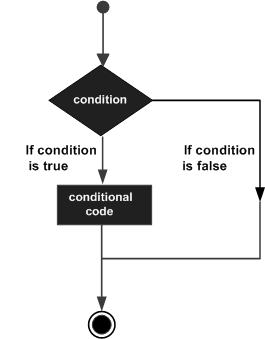MATLAB provides following types of decision making statements. Click the following links to check their detail −

Sr.No. Statement & Description
1 if ... end statement

An if ... end statement consists of a boolean expression followed by one or more statements.

2 if...else...end statement

An if statement can be followed by an optional else statement, which executes when the boolean expression is false.

3 If... elseif...elseif...else...end statements

An if statement can be followed by one (or more) optional elseif... and an else statement, which is very useful to test various conditions.

4 nested if statements

You can use one if or elseif statement inside another if or elseif statement(s).

5 switch statement

A switch statement allows a variable to be tested for equality against a list of values.

6 nested switch statements

You can use one switch statement inside another switch statement(s).

# MATLAB - Loop Types

There may be a situation when you need to execute a block of code several number of times. In general, statements are executed sequentially. The first statement in a function is executed first, followed by the second, and so on.

Programming languages provide various control structures that allow for more complicated execution paths.

A loop statement allows us to execute a statement or group of statements multiple times and following is the general form of a loop statement in most of the programming languages −MATLAB provides following types of loops to handle looping requirements. Click the following links to check their detail −

Sr.No. Loop Type & Description
1 while loop

Repeats a statement or group of statements while a given condition is true. It tests the condition before executing the loop body.

2 for loop

Executes a sequence of statements multiple times and abbreviates the code that manages the loop variable.

3 nested loops

You can use one or more loops inside any another loop.

## Loop Control Statements

Loop control statements change execution from its normal sequence. When execution leaves a scope, all automatic objects that were created in that scope are destroyed.

MATLAB supports the following control statements. Click the following links to check their detail.

Sr.No. Control Statement & Description
1 break statement

Terminates the loop statement and transfers execution to the statement immediately following the loop.

2 continue statement

Causes the loop to skip the remainder of its body and immediately retest its condition prior to reiterating.

# MATLAB - Vectors

A vector is a one-dimensional array of numbers. MATLAB allows creating two types of vectors −

• Row vectors
• Column vectors

## Row Vectors

Row vectors are created by enclosing the set of elements in square brackets, using space or comma to delimit the elements.

```r = [7 8 9 10 11]
```

MATLAB will execute the above statement and return the following result −

```r =

7    8    9   10   11
```

## Column Vectors

Column vectors are created by enclosing the set of elements in square brackets, using semicolon to delimit the elements.

```c = [7;  8;  9;  10; 11]
```

MATLAB will execute the above statement and return the following result −

```c =
7
8
9
10
11

```

## Referencing the Elements of a Vector

You can reference one or more of the elements of a vector in several ways. The ith component of a vector v is referred as v(i). For example −

```v = [ 1; 2; 3; 4; 5; 6];	% creating a column vector of 6 elements
v(3)
```

MATLAB will execute the above statement and return the following result −

```ans =  3
```

When you reference a vector with a colon, such as v(:), all the components of the vector are listed.

```v = [ 1; 2; 3; 4; 5; 6];	% creating a column vector of 6 elements
v(:)
```

MATLAB will execute the above statement and return the following result −

```ans =
1
2
3
4
5
6
```

MATLAB allows you to select a range of elements from a vector.

For example, let us create a row vector rv of 9 elements, then we will reference the elements 3 to 7 by writing rv(3:7) and create a new vector named sub_rv.

```rv = [1 2 3 4 5 6 7 8 9];
sub_rv = rv(3:7)
```

MATLAB will execute the above statement and return the following result −

```sub_rv =

3   4   5   6   7
```

## Vector Operations

In this section, let us discuss the following vector operations −

# MATLAB - Matrix

A matrix is a two-dimensional array of numbers.

In MATLAB, you create a matrix by entering elements in each row as comma or space delimited numbers and using semicolons to mark the end of each row.

For example, let us create a 4-by-5 matrix a

```a = [ 1 2 3 4 5; 2 3 4 5 6; 3 4 5 6 7; 4 5 6 7 8]
```

MATLAB will execute the above statement and return the following result −

```a =
1     2     3     4     5
2     3     4     5     6
3     4     5     6     7
4     5     6     7     8
```

## Referencing the Elements of a Matrix

To reference an element in the mth row and nth column, of a matrix mx, we write −

```mx(m, n);
```

For example, to refer to the element in the 2nd row and 5th column, of the matrix a, as created in the last section, we type −

```a = [ 1 2 3 4 5; 2 3 4 5 6; 3 4 5 6 7; 4 5 6 7 8];
a(2,5)
```

MATLAB will execute the above statement and return the following result −

```ans =  6
```

To reference all the elements in the mth column we type A(:,m).

Let us create a column vector v, from the elements of the 4th row of the matrix a −

```a = [ 1 2 3 4 5; 2 3 4 5 6; 3 4 5 6 7; 4 5 6 7 8];
v = a(:,4)
```

MATLAB will execute the above statement and return the following result −

```v =
4
5
6
7
```

You can also select the elements in the mth through nth columns, for this we write −

```a(:,m:n)
```

Let us create a smaller matrix taking the elements from the second and third columns −

```a = [ 1 2 3 4 5; 2 3 4 5 6; 3 4 5 6 7; 4 5 6 7 8];
a(:, 2:3)
```

MATLAB will execute the above statement and return the following result −

```ans =
2     3
3     4
4     5
5     6
```

In the same way, you can create a sub-matrix taking a sub-part of a matrix.

```a = [ 1 2 3 4 5; 2 3 4 5 6; 3 4 5 6 7; 4 5 6 7 8];
a(:, 2:3)
```

MATLAB will execute the above statement and return the following result −

```ans =
2     3
3     4
4     5
5     6
```

In the same way, you can create a sub-matrix taking a sub-part of a matrix.

For example, let us create a sub-matrix sa taking the inner subpart of a −

```3     4     5
4     5     6
```

To do this, write −

```a = [ 1 2 3 4 5; 2 3 4 5 6; 3 4 5 6 7; 4 5 6 7 8];
sa = a(2:3,2:4)
```

MATLAB will execute the above statement and return the following result −

```sa =
3     4     5
4     5     6
```

## Deleting a Row or a Column in a Matrix

You can delete an entire row or column of a matrix by assigning an empty set of square braces [] to that row or column. Basically, [] denotes an empty array.

For example, let us delete the fourth row of a −

```a = [ 1 2 3 4 5; 2 3 4 5 6; 3 4 5 6 7; 4 5 6 7 8];
a( 4 , : ) = []
```

MATLAB will execute the above statement and return the following result −

```a =
1     2     3     4     5
2     3     4     5     6
3     4     5     6     7
```

Next, let us delete the fifth column of a −

```a = [ 1 2 3 4 5; 2 3 4 5 6; 3 4 5 6 7; 4 5 6 7 8];
a(: , 5)=[]
```

MATLAB will execute the above statement and return the following result −

```a =
1     2     3     4
2     3     4     5
3     4     5     6
4     5     6     7
```

### Example

In this example, let us create a 3-by-3 matrix m, then we will copy the second and third rows of this matrix twice to create a 4-by-3 matrix.

Create a script file with the following code −

```a = [ 1 2 3 ; 4 5 6; 7 8 9];
new_mat = a([2,3,2,3],:)
```

When you run the file, it displays the following result −

```new_mat =
4     5     6
7     8     9
4     5     6
7     8     9
```

## Matrix Operations

In this section, let us discuss the following basic and commonly used matrix operations −

# MATLAB - Arrays

All variables of all data types in MATLAB are multidimensional arrays. A vector is a one-dimensional array and a matrix is a two-dimensional array.

We have already discussed vectors and matrices. In this chapter, we will discuss multidimensional arrays. However, before that, let us discuss some special types of arrays.

## Special Arrays in MATLAB

In this section, we will discuss some functions that create some special arrays. For all these functions, a single argument creates a square array, double arguments create rectangular array.

The zeros() function creates an array of all zeros −

For example −

```zeros(5)
```

MATLAB will execute the above statement and return the following result −

```ans =
0     0     0     0     0
0     0     0     0     0
0     0     0     0     0
0     0     0     0     0
0     0     0     0     0
```

The ones() function creates an array of all ones −

For example −

```ones(4,3)
```

MATLAB will execute the above statement and return the following result −

```ans =
1     1     1
1     1     1
1     1     1
1     1     1
```

The eye() function creates an identity matrix.

For example −

```eye(4)
```

MATLAB will execute the above statement and return the following result −

```ans =
1     0     0     0
0     1     0     0
0     0     1     0
0     0     0     1
```

The rand() function creates an array of uniformly distributed random numbers on (0,1) −

For example −

```rand(3, 5)
```

MATLAB will execute the above statement and return the following result −

```ans =
0.8147    0.9134    0.2785    0.9649    0.9572
0.9058    0.6324    0.5469    0.1576    0.4854
0.1270    0.0975    0.9575    0.9706    0.8003
```

## A Magic Square

A magic square is a square that produces the same sum, when its elements are added row-wise, column-wise or diagonally.

The magic() function creates a magic square array. It takes a singular argument that gives the size of the square. The argument must be a scalar greater than or equal to 3.

```magic(4)
```

MATLAB will execute the above statement and return the following result −

```ans =
16     2     3    13
5    11    10     8
9     7     6    12
4    14    15     1
```

## Multidimensional Arrays

An array having more than two dimensions is called a multidimensional array in MATLAB. Multidimensional arrays in MATLAB are an extension of the normal two-dimensional matrix.

Generally to generate a multidimensional array, we first create a two-dimensional array and extend it.

For example, let's create a two-dimensional array a.

```a = [7 9 5; 6 1 9; 4 3 2]
```

MATLAB will execute the above statement and return the following result −

```a =
7     9     5
6     1     9
4     3     2
```

The array a is a 3-by-3 array; we can add a third dimension to a, by providing the values like −

```a(:, :, 2)= [ 1 2 3; 4 5 6; 7 8 9]
```

MATLAB will execute the above statement and return the following result −

```a =

ans(:,:,1) =

0   0   0
0   0   0
0   0   0

ans(:,:,2) =

1   2   3
4   5   6
7   8   9
```

We can also create multidimensional arrays using the ones(), zeros() or the rand() functions.

For example,

```b = rand(4,3,2)
```

MATLAB will execute the above statement and return the following result −

```b(:,:,1) =
0.0344    0.7952    0.6463
0.4387    0.1869    0.7094
0.3816    0.4898    0.7547
0.7655    0.4456    0.2760

b(:,:,2) =
0.6797    0.4984    0.2238
0.6551    0.9597    0.7513
0.1626    0.3404    0.2551
0.1190    0.5853    0.5060
```

We can also use the cat() function to build multidimensional arrays. It concatenates a list of arrays along a specified dimension −

Syntax for the cat() function is −

```B = cat(dim, A1, A2...)
```

Where,

• B is the new array created

• A1, A2, ... are the arrays to be concatenated

• dim is the dimension along which to concatenate the arrays

### Example

Create a script file and type the following code into it −

```a = [9 8 7; 6 5 4; 3 2 1];
b = [1 2 3; 4 5 6; 7 8 9];
c = cat(3, a, b, [ 2 3 1; 4 7 8; 3 9 0])
```

When you run the file, it displays −

```c(:,:,1) =
9     8     7
6     5     4
3     2     1
c(:,:,2) =
1     2     3
4     5     6
7     8     9
c(:,:,3) =
2     3     1
4     7     8
3     9     0
```

## Array Functions

MATLAB provides the following functions to sort, rotate, permute, reshape, or shift array contents.

Function Purpose
length Length of vector or largest array dimension
ndims Number of array dimensions
numel Number of array elements
size Array dimensions
iscolumn Determines whether input is column vector
isempty Determines whether array is empty
ismatrix Determines whether input is matrix
isrow Determines whether input is row vector
isscalar Determines whether input is scalar
isvector Determines whether input is vector
blkdiag Constructs block diagonal matrix from input arguments
circshift Shifts array circularly
ctranspose Complex conjugate transpose
diag Diagonal matrices and diagonals of matrix
flipdim Flips array along specified dimension
fliplr Flips matrix from left to right
flipud Flips matrix up to down
ipermute Inverses permute dimensions of N-D array
permute Rearranges dimensions of N-D array
repmat Replicates and tile array
reshape Reshapes array
rot90 Rotates matrix 90 degrees
shiftdim Shifts dimensions
issorted Determines whether set elements are in sorted order
sort Sorts array elements in ascending or descending order
sortrows Sorts rows in ascending order
squeeze Removes singleton dimensions
transpose Transpose
vectorize Vectorizes expression

### Examples

The following examples illustrate some of the functions mentioned above.

Length, Dimension and Number of elements −

Create a script file and type the following code into it −

```x = [7.1, 3.4, 7.2, 28/4, 3.6, 17, 9.4, 8.9];
length(x)      % length of x vector
y = rand(3, 4, 5, 2);
ndims(y)       % no of dimensions in array y
s = ['Zara', 'Nuha', 'Shamim', 'Riz', 'Shadab'];
numel(s)       % no of elements in s
```

When you run the file, it displays the following result −

```ans =  8
ans =  4
ans =  23
```

Circular Shifting of the Array Elements −

Create a script file and type the following code into it −

```a = [1 2 3; 4 5 6; 7 8 9]  % the original array a
b = circshift(a,1)         %  circular shift first dimension values down by 1.
c = circshift(a,[1 -1])    % circular shift first dimension values % down by 1
% and second dimension values to the left % by 1.
```

When you run the file, it displays the following result −

```a =
1     2     3
4     5     6
7     8     9

b =
7     8     9
1     2     3
4     5     6

c =
8     9     7
2     3     1
5     6     4
```

## Sorting Arrays

Create a script file and type the following code into it −

```v = [ 23 45 12 9 5 0 19 17]  % horizontal vector
sort(v)                      % sorting v
m = [2 6 4; 5 3 9; 2 0 1]    % two dimensional array
sort(m, 1)                   % sorting m along the row
sort(m, 2)                   % sorting m along the column
```

When you run the file, it displays the following result −

```v =
23    45    12     9     5     0    19    17
ans =
0     5     9    12    17    19    23    45
m =
2     6     4
5     3     9
2     0     1
ans =
2     0     1
2     3     4
5     6     9
ans =
2     4     6
3     5     9
0     1     2
```

## Cell Array

Cell arrays are arrays of indexed cells where each cell can store an array of a different dimensions and data types.

The cell function is used for creating a cell array. Syntax for the cell function is −

```C = cell(dim)
C = cell(dim1,...,dimN)
D = cell(obj)
```

### Where,

• C is the cell array;

• dim is a scalar integer or vector of integers that specifies the dimensions of cell array C;

• dim1, ... , dimN are scalar integers that specify the dimensions of C;

• obj is One of the following −

• Java array or object
• .NET array of type System.String or System.Object

### Example

Create a script file and type the following code into it −

```c = cell(2, 5);
c = {'Red', 'Blue', 'Green', 'Yellow', 'White'; 1 2 3 4 5}
```

When you run the file, it displays the following result −

```c =
{
[1,1] = Red
[2,1] =  1
[1,2] = Blue
[2,2] =  2
[1,3] = Green
[2,3] =  3
[1,4] = Yellow
[2,4] =  4
[1,5] = White
[2,5] =  5
}
```

## Accessing Data in Cell Arrays

There are two ways to refer to the elements of a cell array −

• Enclosing the indices in first bracket (), to refer to sets of cells
• Enclosing the indices in braces {}, to refer to the data within individual cells

When you enclose the indices in first bracket, it refers to the set of cells.

Cell array indices in smooth parentheses refer to sets of cells.

For example −

```c = {'Red', 'Blue', 'Green', 'Yellow', 'White'; 1 2 3 4 5};
c(1:2,1:2)
```

MATLAB will execute the above statement and return the following result −

```ans =
{
[1,1] = Red
[2,1] =  1
[1,2] = Blue
[2,2] =  2
}
```

You can also access the contents of cells by indexing with curly braces.

For example −

```c = {'Red', 'Blue', 'Green', 'Yellow', 'White'; 1 2 3 4 5};
c{1, 2:4}
```

MATLAB will execute the above statement and return the following result −

```ans = Blue
ans = Green
ans = Yellow
```

# MATLAB - Colon Notation

The colon(:) is one of the most useful operator in MATLAB. It is used to create vectors, subscript arrays, and specify for iterations.

If you want to create a row vector, containing integers from 1 to 10, you write −

```1:10
```

MATLAB executes the statement and returns a row vector containing the integers from 1 to 10 −

```ans =

1    2    3    4    5    6    7    8    9   10
```

If you want to specify an increment value other than one, for example −

```100: -5: 50
```

MATLAB executes the statement and returns the following result −

```ans =
100    95    90    85    80    75    70    65    60    55    50
```

Let us take another example −

```0:pi/8:pi
```

MATLAB executes the statement and returns the following result −

```ans =
Columns 1 through 7
0    0.3927    0.7854    1.1781    1.5708    1.9635    2.3562
Columns 8 through 9
2.7489    3.1416
```

You can use the colon operator to create a vector of indices to select rows, columns or elements of arrays.

The following table describes its use for this purpose (let us have a matrix A) −

Format Purpose
A(:,j) is the jth column of A.
A(i,:) is the ith row of A.
A(:,:) is the equivalent two-dimensional array. For matrices this is the same as A.
A(j:k) is A(j), A(j+1),...,A(k).
A(:,j:k) is A(:,j), A(:,j+1),...,A(:,k).
A(:,:,k) is the kth page of three-dimensional array A.
A(i,j,k,:) is a vector in four-dimensional array A. The vector includes A(i,j,k,1), A(i,j,k,2), A(i,j,k,3), and so on.
A(:) is all the elements of A, regarded as a single column. On the left side of an assignment statement, A(:) fills A, preserving its shape from before. In this case, the right side must contain the same number of elements as A.

## Example

Create a script file and type the following code in it −

```A = [1 2 3 4; 4 5 6 7; 7 8 9 10]
A(:,2)      % second column of A
A(:,2:3)    % second and third column of A
A(2:3,2:3)  % second and third rows and second and third columns
```

When you run the file, it displays the following result −

```A =
1     2     3     4
4     5     6     7
7     8     9    10

ans =
2
5
8

ans =
2     3
5     6
8     9

ans =
5     6
8     9
```

# MATLAB - Numbers

MATLAB supports various numeric classes that include signed and unsigned integers and single-precision and double-precision floating-point numbers. By default, MATLAB stores all numeric values as double-precision floating point numbers.

You can choose to store any number or array of numbers as integers or as single-precision numbers.

All numeric types support basic array operations and mathematical operations.

## Conversion to Various Numeric Data Types

MATLAB provides the following functions to convert to various numeric data types −

Function Purpose
double Converts to double precision number
single Converts to single precision number
int8 Converts to 8-bit signed integer
int16 Converts to 16-bit signed integer
int32 Converts to 32-bit signed integer
int64 Converts to 64-bit signed integer
uint8 Converts to 8-bit unsigned integer
uint16 Converts to 16-bit unsigned integer
uint32 Converts to 32-bit unsigned integer
uint64 Converts to 64-bit unsigned integer

### Example

Create a script file and type the following code −

```x = single([5.32 3.47 6.28]) .* 7.5
x = double([5.32 3.47 6.28]) .* 7.5
x = int8([5.32 3.47 6.28]) .* 7.5
x = int16([5.32 3.47 6.28]) .* 7.5
x = int32([5.32 3.47 6.28]) .* 7.5
x = int64([5.32 3.47 6.28]) .* 7.5
```

When you run the file, it shows the following result −

```x =

39.900   26.025   47.100

x =

39.900   26.025   47.100

x =

38  23  45

x =

38  23  45

x =

38  23  45

x =

38  23  45
```

### Example

Let us extend the previous example a little more. Create a script file and type the following code −

```x = int32([5.32 3.47 6.28]) .* 7.5
x = int64([5.32 3.47 6.28]) .* 7.5
x = num2cell(x)
```

When you run the file, it shows the following result −

```x =

38  23  45

x =

38  23  45

x =
{
[1,1] = 38
[1,2] = 23
[1,3] = 45
}
```

## Smallest and Largest Integers

The functions intmax() and intmin() return the maximum and minimum values that can be represented with all types of integer numbers.

Both the functions take the integer data type as the argument, for example, intmax(int8) or intmin(int64) and return the maximum and minimum values that you can represent with the integer data type.

### Example

The following example illustrates how to obtain the smallest and largest values of integers. Create a script file and write the following code in it −

```% displaying the smallest and largest signed integer data
str = 'The range for int8 is:\n\t%d to %d ';
sprintf(str, intmin('int8'), intmax('int8'))
str = 'The range for int16 is:\n\t%d to %d ';
sprintf(str, intmin('int16'), intmax('int16'))
str = 'The range for int32 is:\n\t%d to %d ';
sprintf(str, intmin('int32'), intmax('int32'))
str = 'The range for int64 is:\n\t%d to %d ';
sprintf(str, intmin('int64'), intmax('int64'))

% displaying the smallest and largest unsigned integer data
str = 'The range for uint8 is:\n\t%d to %d ';
sprintf(str, intmin('uint8'), intmax('uint8'))
str = 'The range for uint16 is:\n\t%d to %d ';
sprintf(str, intmin('uint16'), intmax('uint16'))
str = 'The range for uint32 is:\n\t%d to %d ';
sprintf(str, intmin('uint32'), intmax('uint32'))
str = 'The range for uint64 is:\n\t%d to %d ';
sprintf(str, intmin('uint64'), intmax('uint64'))
```

When you run the file, it shows the following result −

```ans = The range for int8 is:
-128 to 127
ans = The range for int16 is:
-32768 to 32767
ans = The range for int32 is:
-2147483648 to 2147483647
ans = The range for int64 is:
0 to 0
ans = The range for uint8 is:
0 to 255
ans = The range for uint16 is:
0 to 65535
ans = The range for uint32 is:
0 to -1
ans = The range for uint64 is:
0 to 18446744073709551616
```

## Smallest and Largest Floating Point Numbers

The functions realmax() and realmin() return the maximum and minimum values that can be represented with floating point numbers.

Both the functions when called with the argument 'single', return the maximum and minimum values that you can represent with the single-precision data type and when called with the argument 'double', return the maximum and minimum values that you can represent with the double-precision data type.

### Example

The following example illustrates how to obtain the smallest and largest floating point numbers. Create a script file and write the following code in it −

```% displaying the smallest and largest single-precision
% floating point number
str = 'The range for single is:\n\t%g to %g and\n\t %g to  %g';
sprintf(str, -realmax('single'), -realmin('single'), ...
realmin('single'), realmax('single'))

% displaying the smallest and largest double-precision
% floating point number
str = 'The range for double is:\n\t%g to %g and\n\t %g to  %g';
sprintf(str, -realmax('double'), -realmin('double'), ...
realmin('double'), realmax('double'))
```

When you run the file, it displays the following result −

```ans = The range for single is:
-3.40282e+38 to -1.17549e-38 and
1.17549e-38 to  3.40282e+38
ans = The range for double is:
-1.79769e+308 to -2.22507e-308 and
2.22507e-308 to  1.79769e+308
```

# MATLAB - Strings

Creating a character string is quite simple in MATLAB. In fact, we have used it many times. For example, you type the following in the command prompt −

```my_string = 'Tutorials Point'
```

MATLAB will execute the above statement and return the following result −

```my_string = Tutorials Point
```

MATLAB considers all variables as arrays, and strings are considered as character arrays. Let us use the whos command to check the variable created above −

```whos
```

MATLAB will execute the above statement and return the following result −

```Name           Size            Bytes  Class    Attributes
my_string      1x16               32  char
```

Interestingly, you can use numeric conversion functions like uint8 or uint16 to convert the characters in the string to their numeric codes. The char function converts the integer vector back to characters −

## Example

Create a script file and type the following code into it −

```my_string = 'Tutorial''s Point';
str_ascii = uint8(my_string)        % 8-bit ascii values
str_back_to_char= char(str_ascii)
str_16bit = uint16(my_string)       % 16-bit ascii values
str_back_to_char = char(str_16bit)
```

When you run the file, it displays the following result −

```str_ascii =

84  117  116  111  114  105   97  108   39  115   32   80  111  105  110  116

str_back_to_char = Tutorial's Point
str_16bit =

84  117  116  111  114  105   97  108   39  115   32   80  111  105  110  116

str_back_to_char = Tutorial's Point
```

## Rectangular Character Array

The strings we have discussed so far are one-dimensional character arrays; however, we need to store more than that. We need to store more dimensional textual data in our program. This is achieved by creating rectangular character arrays.

Simplest way of creating a rectangular character array is by concatenating two or more one-dimensional character arrays, either vertically or horizontally as required.

You can combine strings vertically in either of the following ways −

• Using the MATLAB concatenation operator [] and separating each row with a semicolon (;). Please note that in this method each row must contain the same number of characters. For strings with different lengths, you should pad with space characters as needed.

• Using the char function. If the strings are of different lengths, char pads the shorter strings with trailing blanks so that each row has the same number of characters.

### Example

Create a script file and type the following code into it −

```doc_profile = ['Zara Ali                             '; ...
'Sr. Surgeon                          '; ...
'R N Tagore Cardiology Research Center']
doc_profile = char('Zara Ali', 'Sr. Surgeon', ...
'RN Tagore Cardiology Research Center')
```

When you run the file, it displays the following result −

```doc_profile =
Zara Ali
Sr. Surgeon
R N Tagore Cardiology Research Center
doc_profile =
Zara Ali
Sr. Surgeon
RN Tagore Cardiology Research Center
```

You can combine strings horizontally in either of the following ways −

• Using the MATLAB concatenation operator, [] and separating the input strings with a comma or a space. This method preserves any trailing spaces in the input arrays.

• Using the string concatenation function, strcat. This method removes trailing spaces in the inputs.

### Example

Create a script file and type the following code into it −

```name =     'Zara Ali                             ';
position = 'Sr. Surgeon                          ';
worksAt =  'R N Tagore Cardiology Research Center';
profile = [name ', ' position ', ' worksAt]
profile = strcat(name, ', ', position, ', ', worksAt)
```

When you run the file, it displays the following result −

```profile = Zara Ali      , Sr. Surgeon      , R N Tagore Cardiology Research Center
profile = Zara Ali,Sr. Surgeon,R N Tagore Cardiology Research Center
```

## Combining Strings into a Cell Array

From our previous discussion, it is clear that combining strings with different lengths could be a pain as all strings in the array has to be of the same length. We have used blank spaces at the end of strings to equalize their length.

However, a more efficient way to combine the strings is to convert the resulting array into a cell array.

MATLAB cell array can hold different sizes and types of data in an array. Cell arrays provide a more flexible way to store strings of varying length.

The cellstr function converts a character array into a cell array of strings.

### Example

Create a script file and type the following code into it −

```name =     'Zara Ali                             ';
position = 'Sr. Surgeon                          ';
worksAt =  'R N Tagore Cardiology Research Center';
profile = char(name, position, worksAt);
profile = cellstr(profile);
disp(profile)
```

When you run the file, it displays the following result −

```{
[1,1] = Zara Ali
[2,1] = Sr. Surgeon
[3,1] = R N Tagore Cardiology Research Center
}
```

## String Functions in MATLAB

MATLAB provides numerous string functions creating, combining, parsing, comparing and manipulating strings.

Following table provides brief description of the string functions in MATLAB −

Function Purpose
Functions for storing text in character arrays, combine character arrays, etc.
blanks Create string of blank characters
cellstr Create cell array of strings from character array
char Convert to character array (string)
iscellstr Determine whether input is cell array of strings
ischar Determine whether item is character array
sprintf Format data into string
strcat Concatenate strings horizontally
strjoin Join strings in cell array into single string
Functions for identifying parts of strings, find and replace substrings
ischar Determine whether item is character array
isletter Array elements that are alphabetic letters
isspace Array elements that are space characters
isstrprop Determine whether string is of specified category
sscanf Read formatted data from string
strfind Find one string within another
strrep Find and replace substring
strsplit Split string at specified delimiter
strtok Selected parts of string
validatestring Check validity of text string
symvar Determine symbolic variables in expression
regexp Match regular expression (case sensitive)
regexpi Match regular expression (case insensitive)
regexprep Replace string using regular expression
regexptranslate Translate string into regular expression
Functions for string comparison
strcmp Compare strings (case sensitive)
strcmpi Compare strings (case insensitive)
strncmp Compare first n characters of strings (case sensitive)
strncmpi Compare first n characters of strings (case insensitive)
Functions for changing string to upper- or lowercase, creating or removing white space
deblank Strip trailing blanks from end of string
strtrim Remove leading and trailing white space from string
lower Convert string to lowercase
upper Convert string to uppercase
strjust Justify character array

### Examples

The following examples illustrate some of the above-mentioned string functions −

#### Formatting Strings

Create a script file and type the following code into it −

```A = pi*1000*ones(1,5);
sprintf(' %f \n %.2f \n %+.2f \n %12.2f \n %012.2f \n', A)
```

When you run the file, it displays the following result −

```ans =  3141.592654
3141.59
+3141.59
3141.59
000003141.59
```

#### Joining Strings

Create a script file and type the following code into it −

```%cell array of strings
str_array = {'red','blue','green', 'yellow', 'orange'};

% Join strings in cell array into single string
str1 = strjoin(str_array, "-")
str2 = strjoin(str_array, ",")
```

When you run the file, it displays the following result −

```str1 = red-blue-green-yellow-orange
str2 = red,blue,green,yellow,orange
```

#### Finding and Replacing Strings

Create a script file and type the following code into it −

```students = {'Zara Ali', 'Neha Bhatnagar', ...
'Monica Malik', 'Madhu Gautam', ...
'Madhu Sharma', 'Bhawna Sharma',...
'Nuha Ali', 'Reva Dutta', ...
'Sunaina Ali', 'Sofia Kabir'};

% The strrep function searches and replaces sub-string.
new_student = strrep(students(8), 'Reva', 'Poulomi')
% Display first names
first_names = strtok(students)
```

When you run the file, it displays the following result −

```new_student =
{
[1,1] = Poulomi Dutta
}
first_names =
{
[1,1] = Zara
[1,2] = Neha
[1,3] = Monica
[1,6] = Bhawna
[1,7] = Nuha
[1,8] = Reva
[1,9] = Sunaina
[1,10] = Sofia
}
```

#### Comparing Strings

Create a script file and type the following code into it −

```str1 = 'This is test'
str2 = 'This is text'
if (strcmp(str1, str2))
sprintf('%s and %s are equal', str1, str2)
else
sprintf('%s and %s are not equal', str1, str2)
end
```

When you run the file, it displays the following result −

```str1 = This is test
str2 = This is text
ans = This is test and This is text are not equal
```

# MATLAB - Functions

A function is a group of statements that together perform a task. In MATLAB, functions are defined in separate files. The name of the file and of the function should be the same.

Functions operate on variables within their own workspace, which is also called the local workspace, separate from the workspace you access at the MATLAB command prompt which is called the base workspace.

Functions can accept more than one input arguments and may return more than one output arguments.

Syntax of a function statement is −

```function [out1,out2, ..., outN] = myfun(in1,in2,in3, ..., inN)
```

## Example

The following function named mymax should be written in a file named mymax.m. It takes five numbers as argument and returns the maximum of the numbers.

Create a function file, named mymax.m and type the following code in it −

```function max = mymax(n1, n2, n3, n4, n5)

%This function calculates the maximum of the
% five numbers given as input
max =  n1;
if(n2 > max)
max = n2;
end
if(n3 > max)
max = n3;
end
if(n4 > max)
max = n4;
end
if(n5 > max)
max = n5;
end
```

The first line of a function starts with the keyword function. It gives the name of the function and order of arguments. In our example, the mymax function has five input arguments and one output argument.

The comment lines that come right after the function statement provide the help text. These lines are printed when you type −

```help mymax
```

MATLAB will execute the above statement and return the following result −

```This function calculates the maximum of the
five numbers given as input
```

You can call the function as −

```mymax(34, 78, 89, 23, 11)
```

MATLAB will execute the above statement and return the following result −

```ans = 89
```

## Anonymous Functions

An anonymous function is like an inline function in traditional programming languages, defined within a single MATLAB statement. It consists of a single MATLAB expression and any number of input and output arguments.

You can define an anonymous function right at the MATLAB command line or within a function or script.

This way you can create simple functions without having to create a file for them.

The syntax for creating an anonymous function from an expression is

```f = @(arglist)expression
```

### Example

In this example, we will write an anonymous function named power, which will take two numbers as input and return first number raised to the power of the second number.

Create a script file and type the following code in it −

```power = @(x, n) x.^n;
result1 = power(7, 3)
result2 = power(49, 0.5)
result3 = power(10, -10)
result4 = power (4.5, 1.5)
```

When you run the file, it displays −

```result1 =  343
result2 =  7
result3 =  1.0000e-10
result4 =  9.5459
```

## Primary and Sub-Functions

Any function other than an anonymous function must be defined within a file. Each function file contains a required primary function that appears first and any number of optional sub-functions that comes after the primary function and used by it.

Primary functions can be called from outside of the file that defines them, either from command line or from other functions, but sub-functions cannot be called from command line or other functions, outside the function file.

Sub-functions are visible only to the primary function and other sub-functions within the function file that defines them.

### Example

Let us write a function named quadratic that would calculate the roots of a quadratic equation. The function would take three inputs, the quadratic co-efficient, the linear co-efficient and the constant term. It would return the roots.

The function file quadratic.m will contain the primary function quadratic and the sub-function disc, which calculates the discriminant.

Create a function file quadratic.m and type the following code in it −

```function [x1,x2] = quadratic(a,b,c)

%this function returns the roots of
% a quadratic equation.
% It takes 3 input arguments
% which are the co-efficients of x2, x and the
%constant term
% It returns the roots
d = disc(a,b,c);
x1 = (-b + d) / (2*a);
x2 = (-b - d) / (2*a);
end   % end of quadratic

function dis = disc(a,b,c)
%function calculates the discriminant
dis = sqrt(b^2 - 4*a*c);
end   % end of sub-function
```

You can call the above function from command prompt as −

```quadratic(2,4,-4)
```

MATLAB will execute the above statement and return the following result −

```ans = 0.7321
```

## Nested Functions

You can define functions within the body of another function. These are called nested functions. A nested function contains any or all of the components of any other function.

Nested functions are defined within the scope of another function and they share access to the containing function's workspace.

A nested function follows the following syntax −

```function x = A(p1, p2)
...
B(p2)
function y = B(p3)
...
end
...
end
```

### Example

Let us rewrite the function quadratic, from previous example, however, this time the disc function will be a nested function.

Create a function file quadratic2.m and type the following code in it −

```function [x1,x2] = quadratic2(a,b,c)
function disc  % nested function
d = sqrt(b^2 - 4*a*c);
end   % end of function disc

disc;
x1 = (-b + d) / (2*a);
x2 = (-b - d) / (2*a);
end   % end of function quadratic2
```

You can call the above function from command prompt as −

```quadratic2(2,4,-4)
```

MATLAB will execute the above statement and return the following result −

```ans =  0.73205
```

## Private Functions

A private function is a primary function that is visible only to a limited group of other functions. If you do not want to expose the implementation of a function(s), you can create them as private functions.

Private functions reside in subfolders with the special name private.

They are visible only to functions in the parent folder.

### Example

Let us rewrite the quadratic function. This time, however, the disc function calculating the discriminant, will be a private function.

Create a subfolder named private in working directory. Store the following function file disc.m in it −

```function dis = disc(a,b,c)
%function calculates the discriminant
dis = sqrt(b^2 - 4*a*c);
end      % end of sub-function
```

Create a function quadratic3.m in your working directory and type the following code in it −

```function [x1,x2] = quadratic3(a,b,c)

%this function returns the roots of
% a quadratic equation.
% It takes 3 input arguments
% which are the co-efficient of x2, x and the
%constant term
% It returns the roots
d = disc(a,b,c);

x1 = (-b + d) / (2*a);
x2 = (-b - d) / (2*a);
end      % end of quadratic3
```

You can call the above function from command prompt as −

```quadratic3(2,4,-4)
```

MATLAB will execute the above statement and return the following result −

```ans =  0.73205
```

## Global Variables

Global variables can be shared by more than one function. For this, you need to declare the variable as global in all the functions.

If you want to access that variable from the base workspace, then declare the variable at the command line.

The global declaration must occur before the variable is actually used in a function. It is a good practice to use capital letters for the names of global variables to distinguish them from other variables.

### Example

Let us create a function file named average.m and type the following code in it −

```function avg = average(nums)
global TOTAL
avg = sum(nums)/TOTAL;
end
```

Create a script file and type the following code in it −

```global TOTAL;
TOTAL = 10;
n = [34, 45, 25, 45, 33, 19, 40, 34, 38, 42];
av = average(n)
```

When you run the file, it will display the following result −

```av =  35.500
```

# MATLAB - Data Import

Importing data in MATLAB means loading data from an external file. The importdata function allows loading various data files of different formats. It has the following five forms −

Sr.No. Function & Description
1

A = importdata(filename)

Loads data into array A from the file denoted by filename.

2

A = importdata('-pastespecial')

Loads data from the system clipboard rather than from a file.

3

A = importdata(___, delimiterIn)

Interprets delimiterIn as the column separator in ASCII file, filename, or the clipboard data. You can use delimiterIn with any of the input arguments in the above syntaxes.

4

A = importdata(___, delimiterIn, headerlinesIn)

Loads data from ASCII file, filename, or the clipboard, reading numeric data starting from line headerlinesIn+1.

5

[A, delimiterOut, headerlinesOut] = importdata(___)

Returns the detected delimiter character for the input ASCII file in delimiterOut and the detected number of header lines in headerlinesOut, using any of the input arguments in the previous syntaxes.

By default, Octave does not have support for importdata() function, so you will have to search and install this package to make following examples work with your Octave installation.

## Example 1

Let us load and display an image file. Create a script file and type the following code in it −

```filename = 'smile.jpg';
A = importdata(filename);
image(A);
```

When you run the file, MATLAB displays the image file. However, you must store it in the current directory.## Example 2

In this example, we import a text file and specify Delimiter and Column Header. Let us create a space-delimited ASCII file with column headers, named weeklydata.txt.

Our text file weeklydata.txt looks like this −

```SunDay  MonDay  TuesDay  WednesDay  ThursDay  FriDay  SaturDay
95.01   76.21   61.54    40.57       55.79    70.28   81.53
73.11   45.65   79.19    93.55       75.29    69.87   74.68
60.68   41.85   92.18    91.69       81.32    90.38   74.51
48.60   82.14   73.82    41.03       0.99     67.22   93.18
89.13   44.47   57.63    89.36       13.89    19.88   46.60
```

Create a script file and type the following code in it −

```filename = 'weeklydata.txt';
delimiterIn = ' ';

% View data
for k = [1:7]
disp(A.data(:, k))
disp(' ')
end
```

When you run the file, it displays the following result −

```SunDay
95.0100
73.1100
60.6800
48.6000
89.1300

MonDay
76.2100
45.6500
41.8500
82.1400
44.4700

TuesDay
61.5400
79.1900
92.1800
73.8200
57.6300

WednesDay
40.5700
93.5500
91.6900
41.0300
89.3600

ThursDay
55.7900
75.2900
81.3200
0.9900
13.8900

FriDay
70.2800
69.8700
90.3800
67.2200
19.8800

SaturDay
81.5300
74.6800
74.5100
93.1800
46.6000
```

## Example 3

In this example, let us import data from clipboard.

Copy the following lines to the clipboard −

Mathematics is simple

Create a script file and type the following code −

```A = importdata('-pastespecial')
```

When you run the file, it displays the following result −

```A =
'Mathematics is simple'
```

## Low-Level File I/O

The importdata function is a high-level function. The low-level file I/O functions in MATLAB allow the most control over reading or writing data to a file. However, these functions need more detailed information about your file to work efficiently.

MATLAB provides the following functions for read and write operations at the byte or character level −

Function Description
fclose Close one or all open files
feof Test for end-of-file
ferror Information about file I/O errors
fgetl Read line from file, removing newline characters
fgets Read line from file, keeping newline characters
fopen Open file, or obtain information about open files
fprintf Write data to text file
frewind Move file position indicator to beginning of open file
fscanf Read data from text file
fseek Move to specified position in file
ftell Position in open file
fwrite Write data to binary file

## Import Text Data Files with Low-Level I/O

MATLAB provides the following functions for low-level import of text data files −

• The fscanf function reads formatted data in a text or ASCII file.

• The fgetl and fgets functions read one line of a file at a time, where a newline character separates each line.

• The fread function reads a stream of data at the byte or bit level.

## Example

We have a text data file 'myfile.txt' saved in our working directory. The file stores rainfall data for three months; June, July and August for the year 2012.

The data in myfile.txt contains repeated sets of time, month and rainfall measurements at five places. The header data stores the number of months M; so we have M sets of measurements.

The file looks like this −

```Rainfall Data
Months: June, July, August

M = 3
12:00:00
June-2012
17.21  28.52  39.78  16.55 23.67
19.15  0.35   17.57  NaN   12.01
17.92  28.49  17.40  17.06 11.09
9.59   9.33   NaN    0.31  0.23
10.46  13.17  NaN    14.89 19.33
20.97  19.50  17.65  14.45 14.00
18.23  10.34  17.95  16.46 19.34
09:10:02
July-2012
12.76  16.94  14.38  11.86 16.89
20.46  23.17  NaN    24.89 19.33
30.97  49.50  47.65  24.45 34.00
18.23  30.34  27.95  16.46 19.34
30.46  33.17  NaN    34.89  29.33
30.97  49.50  47.65  24.45 34.00
28.67  30.34  27.95  36.46 29.34
15:03:40
August-2012
17.09  16.55  19.59  17.25 19.22
17.54  11.45  13.48  22.55 24.01
NaN    21.19  25.85  25.05 27.21
26.79  24.98  12.23  16.99 18.67
17.54  11.45  13.48  22.55 24.01
NaN    21.19  25.85  25.05 27.21
26.79  24.98  12.23  16.99 18.67
```

We will import data from this file and display this data. Take the following steps −

• Open the file with fopen function and get the file identifier.

• Describe the data in the file with format specifiers, such as '%s' for a string, '%d' for an integer, or '%f' for a floating-point number.

• To skip literal characters in the file, include them in the format description. To skip a data field, use an asterisk ('*') in the specifier.

For example, to read the headers and return the single value for M, we write −

```M = fscanf(fid, '%*s %*s\n%*s %*s %*s %*s\nM=%d\n\n', 1);
```
• By default, fscanf reads data according to our format description until it does not find any match for the data, or it reaches the end of the file. Here we will use for loop for reading 3 sets of data and each time, it will read 7 rows and 5 columns.

• We will create a structure named mydata in the workspace to store data read from the file. This structure has three fields - time, month, and raindata array.

Create a script file and type the following code in it −

```filename = '/data/myfile.txt';
rows = 7;
cols = 5;

% open the file
fid = fopen(filename);

% read the file headers, find M (number of months)
M = fscanf(fid, '%*s %*s\n%*s %*s %*s %*s\nM=%d\n\n', 1);

% read each set of measurements
for n = 1:M
mydata(n).time = fscanf(fid, '%s', 1);
mydata(n).month = fscanf(fid, '%s', 1);

% fscanf fills the array in column order,
% so transpose the results
mydata(n).raindata  = ...
fscanf(fid, '%f', [rows, cols]);
end
for n = 1:M
disp(mydata(n).time), disp(mydata(n).month)
disp(mydata(n).raindata)
end

% close the file
fclose(fid);
```

When you run the file, it displays the following result −

```12:00:00
June-2012
17.2100   17.5700   11.0900   13.1700   14.4500
28.5200       NaN    9.5900       NaN   14.0000
39.7800   12.0100    9.3300   14.8900   18.2300
16.5500   17.9200       NaN   19.3300   10.3400
23.6700   28.4900    0.3100   20.9700   17.9500
19.1500   17.4000    0.2300   19.5000   16.4600
0.3500   17.0600   10.4600   17.6500   19.3400

09:10:02
July-2012
12.7600       NaN   34.0000   33.1700   24.4500
16.9400   24.8900   18.2300       NaN   34.0000
14.3800   19.3300   30.3400   34.8900   28.6700
11.8600   30.9700   27.9500   29.3300   30.3400
16.8900   49.5000   16.4600   30.9700   27.9500
20.4600   47.6500   19.3400   49.5000   36.4600
23.1700   24.4500   30.4600   47.6500   29.3400

15:03:40
August-2012
17.0900   13.4800   27.2100   11.4500   25.0500
16.5500   22.5500   26.7900   13.4800   27.2100
19.5900   24.0100   24.9800   22.5500   26.7900
17.2500       NaN   12.2300   24.0100   24.9800
19.2200   21.1900   16.9900       NaN   12.2300
17.5400   25.8500   18.6700   21.1900   16.9900
11.4500   25.0500   17.5400   25.8500   18.6700
```

# MATLAB - Data Output

Data export (or output) in MATLAB means to write into files. MATLAB allows you to use your data in another application that reads ASCII files. For this, MATLAB provides several data export options.

You can create the following type of files −

• Rectangular, delimited ASCII data file from an array.

• Diary (or log) file of keystrokes and the resulting text output.

• Specialized ASCII file using low-level functions such as fprintf.

• MEX-file to access your C/C++ or Fortran routine that writes to a particular text file format.

Apart from this, you can also export data to spreadsheets.

There are two ways to export a numeric array as a delimited ASCII data file −

• Using the save function and specifying the -ascii qualifier

• Using the dlmwrite function

Syntax for using the save function is −

```save my_data.out num_array -ascii
```

where, my_data.out is the delimited ASCII data file created, num_array is a numeric array and −ascii is the specifier.

Syntax for using the dlmwrite function is −

```dlmwrite('my_data.out', num_array, 'dlm_char')
```

where, my_data.out is the delimited ASCII data file created, num_array is a numeric array and dlm_char is the delimiter character.

## Example

The following example demonstrates the concept. Create a script file and type the following code −

```num_array = [ 1 2 3 4 ; 4 5 6 7; 7 8 9 0];
save array_data1.out num_array -ascii;
type array_data1.out
dlmwrite('array_data2.out', num_array, ' ');
type array_data2.out
```

When you run the file, it displays the following result −

```   1.0000000e+00   2.0000000e+00   3.0000000e+00   4.0000000e+00
4.0000000e+00   5.0000000e+00   6.0000000e+00   7.0000000e+00
7.0000000e+00   8.0000000e+00   9.0000000e+00   0.0000000e+00

1 2 3 4
4 5 6 7
7 8 9 0
```

Please note that the save -ascii command and the dlmwrite function does not work with cell arrays as input. To create a delimited ASCII file from the contents of a cell array, you can

• Either, convert the cell array to a matrix using the cell2mat function

• Or export the cell array using low-level file I/O functions.

If you use the save function to write a character array to an ASCII file, it writes the ASCII equivalent of the characters to the file.

For example, let us write the word 'hello' to a file −

```h = 'hello';
save textdata.out h -ascii
type textdata.out
```

MATLAB executes the above statements and displays the following result. which is the characters of the string 'hello' in 8-digit ASCII format.

```1.0400000e+02   1.0100000e+02   1.0800000e+02   1.0800000e+02   1.1100000e+02
```

## Writing to Diary Files

Diary files are activity logs of your MATLAB session. The diary function creates an exact copy of your session in a disk file, excluding graphics.

To turn on the diary function, type −

```diary
```

Optionally, you can give the name of the log file, say −

```diary logdata.out
```

To turn off the diary function −

```diary off
```

You can open the diary file in a text editor.

## Exporting Data to Text Data Files with Low-Level I/O

So far, we have exported numeric arrays. However, you may need to create other text files, including combinations of numeric and character data, nonrectangular output files, or files with non-ASCII encoding schemes. For these purposes, MATLAB provides the low-level fprintf function.

As in low-level I/O file activities, before exporting, you need to open or create a file with the fopen function and get the file identifier. By default, fopen opens a file for read-only access. You should specify the permission to write or append, such as 'w' or 'a'.

After processing the file, you need to close it with fclose(fid) function.

The following example demonstrates the concept −

### Example

Create a script file and type the following code in it −

```% create a matrix y, with two rows
x = 0:10:100;
y = [x; log(x)];

% open a file for writing
fid = fopen('logtable.txt', 'w');

fprintf(fid, 'Log     Function\n\n');

% print values in column order
% two values appear on each row of the file
fprintf(fid, '%f    %f\n', y);
fclose(fid);

% display the file created
type logtable.txt
```

When you run the file, it displays the following result −

```Log         Function

0.000000    -Inf
10.000000    2.302585
20.000000    2.995732
30.000000    3.401197
40.000000    3.688879
50.000000    3.912023
60.000000    4.094345
70.000000    4.248495
80.000000    4.382027
90.000000    4.499810
100.000000    4.605170
```

# MATLAB - Plotting

To plot the graph of a function, you need to take the following steps −

• Define x, by specifying the range of values for the variable x, for which the function is to be plotted

• Define the function, y = f(x)

• Call the plot command, as plot(x, y)

Following example would demonstrate the concept. Let us plot the simple function y = x for the range of values for x from 0 to 100, with an increment of 5.

Create a script file and type the following code −

```x = [0:5:100];
y = x;
plot(x, y)
```

When you run the file, MATLAB displays the following plot −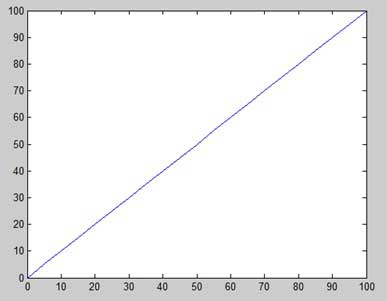Let us take one more example to plot the function y = x2. In this example, we will draw two graphs with the same function, but in second time, we will reduce the value of increment. Please note that as we decrease the increment, the graph becomes smoother.

Create a script file and type the following code −

```x = [1 2 3 4 5 6 7 8 9 10];
x = [-100:20:100];
y = x.^2;
plot(x, y)
```

When you run the file, MATLAB displays the following plot −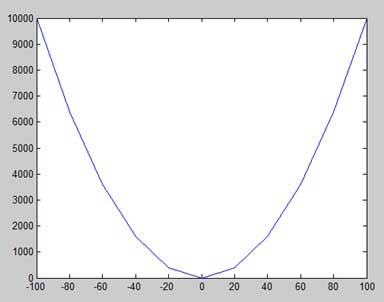Change the code file a little, reduce the increment to 5 −

```x = [-100:5:100];
y = x.^2;
plot(x, y)
```

MATLAB draws a smoother graph −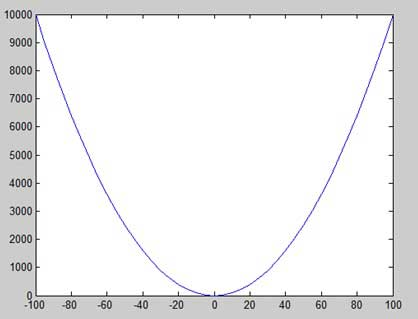## Adding Title, Labels, Grid Lines and Scaling on the Graph

MATLAB allows you to add title, labels along the x-axis and y-axis, grid lines and also to adjust the axes to spruce up the graph.

• The xlabel and ylabel commands generate labels along x-axis and y-axis.

• The title command allows you to put a title on the graph.

• The grid on command allows you to put the grid lines on the graph.

• The axis equal command allows generating the plot with the same scale factors and the spaces on both axes.

• The axis square command generates a square plot.

### Example

Create a script file and type the following code −

```x = [0:0.01:10];
y = sin(x);
plot(x, y), xlabel('x'), ylabel('Sin(x)'), title('Sin(x) Graph'),
grid on, axis equal
```

MATLAB generates the following graph −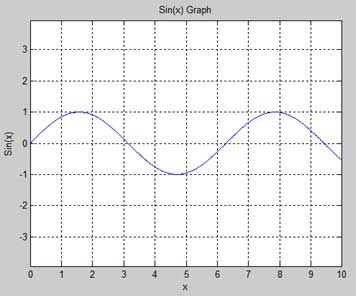## Drawing Multiple Functions on the Same Graph

You can draw multiple graphs on the same plot. The following example demonstrates the concept −

### Example

Create a script file and type the following code −

```x = [0 : 0.01: 10];
y = sin(x);
g = cos(x);
plot(x, y, x, g, '.-'), legend('Sin(x)', 'Cos(x)')
```

MATLAB generates the following graph −## Setting Colors on Graph

MATLAB provides eight basic color options for drawing graphs. The following table shows the colors and their codes −

Code Color
w White
k Black
b Blue
r Red
c Cyan
g Green
m Magenta
y Yellow

### Example

Let us draw the graph of two polynomials

• f(x) = 3x4 + 2x3+ 7x2 + 2x + 9 and

• g(x) = 5x3 + 9x + 2

Create a script file and type the following code −

```x = [-10 : 0.01: 10];
y = 3*x.^4 + 2 * x.^3 + 7 * x.^2 + 2 * x + 9;
g = 5 * x.^3 + 9 * x + 2;
plot(x, y, 'r', x, g, 'g')
```

When you run the file, MATLAB generates the following graph −## Setting Axis Scales

The axis command allows you to set the axis scales. You can provide minimum and maximum values for x and y axes using the axis command in the following way −

```axis ( [xmin xmax ymin ymax] )
```

The following example shows this −

### Example

Create a script file and type the following code −

```x = [0 : 0.01: 10];
y = exp(-x).* sin(2*x + 3);
plot(x, y), axis([0 10 -1 1])
```

When you run the file, MATLAB generates the following graph −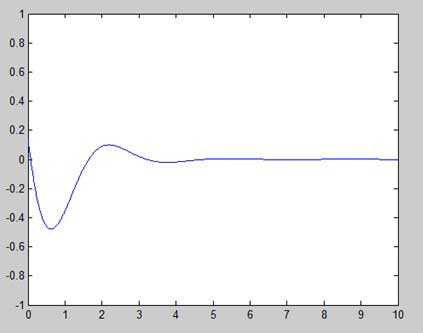## Generating Sub-Plots

When you create an array of plots in the same figure, each of these plots is called a subplot. The subplot command is used for creating subplots.

Syntax for the command is −

```subplot(m, n, p)
```

where, m and n are the number of rows and columns of the plot array and p specifies where to put a particular plot.

Each plot created with the subplot command can have its own characteristics. Following example demonstrates the concept −

### Example

Let us generate two plots −

y = e−1.5xsin(10x)

y = e−2xsin(10x)

Create a script file and type the following code −

```x = [0:0.01:5];
y = exp(-1.5*x).*sin(10*x);
subplot(1,2,1)
plot(x,y), xlabel('x'),ylabel('exp(–1.5x)*sin(10x)'),axis([0 5 -1 1])
y = exp(-2*x).*sin(10*x);
subplot(1,2,2)
plot(x,y),xlabel('x'),ylabel('exp(–2x)*sin(10x)'),axis([0 5 -1 1])
```

When you run the file, MATLAB generates the following graph −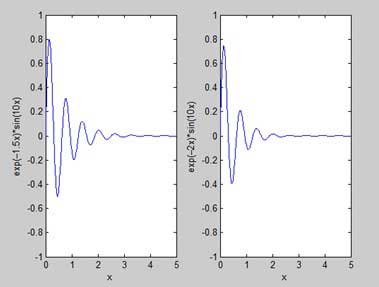# MATLAB - Graphics

This chapter will continue exploring the plotting and graphics capabilities of MATLAB. We will discuss −

• Drawing bar charts
• Drawing contours
• Three dimensional plots

## Drawing Bar Charts

The bar command draws a two dimensional bar chart. Let us take up an example to demonstrate the idea.

### Example

Let us have an imaginary classroom with 10 students. We know the percent of marks obtained by these students are 75, 58, 90, 87, 50, 85, 92, 75, 60 and 95. We will draw the bar chart for this data.

Create a script file and type the following code −

```x = [1:10];
y = [75, 58, 90, 87, 50, 85, 92, 75, 60, 95];
bar(x,y), xlabel('Student'),ylabel('Score'),
title('First Sem:')
print -deps graph.eps
```

When you run the file, MATLAB displays the following bar chart −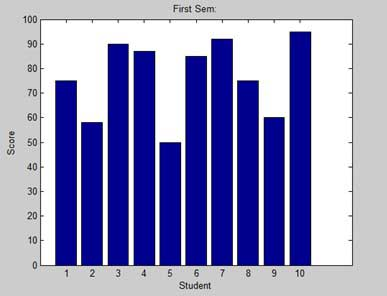## Drawing Contours

A contour line of a function of two variables is a curve along which the function has a constant value. Contour lines are used for creating contour maps by joining points of equal elevation above a given level, such as mean sea level.

MATLAB provides a contour function for drawing contour maps.

### Example

Let us generate a contour map that shows the contour lines for a given function g = f(x, y). This function has two variables. So, we will have to generate two independent variables, i.e., two data sets x and y. This is done by calling the meshgrid command.

The meshgrid command is used for generating a matrix of elements that give the range over x and y along with the specification of increment in each case.

Let us plot our function g = f(x, y), where −5 ≤ x ≤ 5, −3 ≤ y ≤ 3. Let us take an increment of 0.1 for both the values. The variables are set as −

```[x,y] = meshgrid(–5:0.1:5, –3:0.1:3);
```

Lastly, we need to assign the function. Let our function be: x2 + y2

Create a script file and type the following code −

```[x,y] = meshgrid(-5:0.1:5,-3:0.1:3);   %independent variables
g = x.^2 + y.^2;                       % our function
contour(x,y,g)                         % call the contour function
print -deps graph.eps
```

When you run the file, MATLAB displays the following contour map −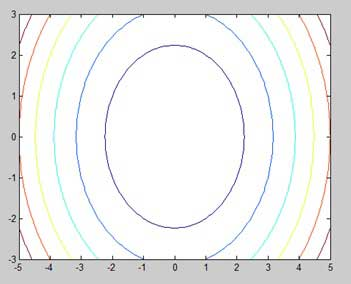Let us modify the code a little to spruce up the map

```[x,y] = meshgrid(-5:0.1:5,-3:0.1:3);   %independent variables
g = x.^2 + y.^2;                       % our function
[C, h] = contour(x,y,g);               % call the contour function
set(h,'ShowText','on','TextStep',get(h,'LevelStep')*2)
print -deps graph.eps
```

When you run the file, MATLAB displays the following contour map −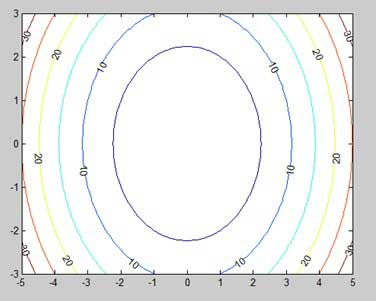## Three Dimensional Plots

Three-dimensional plots basically display a surface defined by a function in two variables, g = f (x,y).

As before, to define g, we first create a set of (x,y) points over the domain of the function using the meshgrid command. Next, we assign the function itself. Finally, we use the surf command to create a surface plot.

The following example demonstrates the concept −

### Example

Let us create a 3D surface map for the function g = xe-(x2 + y2)

Create a script file and type the following code −

```[x,y] = meshgrid(-2:.2:2);
g = x .* exp(-x.^2 - y.^2);
surf(x, y, g)
print -deps graph.eps
```

When you run the file, MATLAB displays the following 3-D map −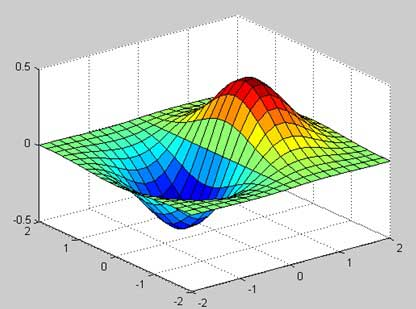You can also use the mesh command to generate a three-dimensional surface. However, the surf command displays both the connecting lines and the faces of the surface in color, whereas, the mesh command creates a wireframe surface with colored lines connecting the defining points.

# MATLAB - Algebra

So far, we have seen that all the examples work in MATLAB as well as its GNU, alternatively called Octave. But for solving basic algebraic equations, both MATLAB and Octave are little different, so we will try to cover MATLAB and Octave in separate sections.

We will also discuss factorizing and simplification of algebraic expressions.

## Solving Basic Algebraic Equations in MATLAB

The solve function is used for solving algebraic equations. In its simplest form, the solve function takes the equation enclosed in quotes as an argument.

For example, let us solve for x in the equation x-5 = 0

```solve('x-5=0')
```

MATLAB will execute the above statement and return the following result −

```ans =
5
```

You can also call the solve function as −

```y = solve('x-5 = 0')
```

MATLAB will execute the above statement and return the following result −

```y =
5
```

You may even not include the right hand side of the equation −

```solve('x-5')
```

MATLAB will execute the above statement and return the following result −

```ans =
5
```

If the equation involves multiple symbols, then MATLAB by default assumes that you are solving for x, however, the solve function has another form −

```solve(equation, variable)
```

where, you can also mention the variable.

For example, let us solve the equation v – u – 3t2 = 0, for v. In this case, we should write −

```solve('v-u-3*t^2=0', 'v')
```

MATLAB will execute the above statement and return the following result −

```ans =
3*t^2 + u
```

## Solving Basic Algebraic Equations in Octave

The roots function is used for solving algebraic equations in Octave and you can write above examples as follows −

For example, let us solve for x in the equation x-5 = 0

```roots([1, -5])
```

Octave will execute the above statement and return the following result −

```ans = 5
```

You can also call the solve function as −

```y = roots([1, -5])
```

Octave will execute the above statement and return the following result −

```y = 5
```

## Solving Quadratic Equations in MATLAB

The solve function can also solve higher order equations. It is often used to solve quadratic equations. The function returns the roots of the equation in an array.

The following example solves the quadratic equation x2 -7x +12 = 0. Create a script file and type the following code −

```eq = 'x^2 -7*x + 12 = 0';
s = solve(eq);
disp('The first root is: '), disp(s(1));
disp('The second root is: '), disp(s(2));
```

When you run the file, it displays the following result −

```The first root is:
3
The second root is:
4
```

## Solving Quadratic Equations in Octave

The following example solves the quadratic equation x2 -7x +12 = 0 in Octave. Create a script file and type the following code −

```s = roots([1, -7, 12]);

disp('The first root is: '), disp(s(1));
disp('The second root is: '), disp(s(2));
```

When you run the file, it displays the following result −

```The first root is:
4
The second root is:
3
```

## Solving Higher Order Equations in MATLAB

The solve function can also solve higher order equations. For example, let us solve a cubic equation as (x-3)2(x-7) = 0

```solve('(x-3)^2*(x-7)=0')
```

MATLAB will execute the above statement and return the following result −

```ans =
3
3
7
```

In case of higher order equations, roots are long containing many terms. You can get the numerical value of such roots by converting them to double. The following example solves the fourth order equation x4 − 7x3 + 3x2 − 5x + 9 = 0.

Create a script file and type the following code −

```eq = 'x^4 - 7*x^3 + 3*x^2 - 5*x + 9 = 0';
s = solve(eq);
disp('The first root is: '), disp(s(1));
disp('The second root is: '), disp(s(2));
disp('The third root is: '), disp(s(3));
disp('The fourth root is: '), disp(s(4));

% converting the roots to double type
disp('Numeric value of first root'), disp(double(s(1)));
disp('Numeric value of second root'), disp(double(s(2)));
disp('Numeric value of third root'), disp(double(s(3)));
disp('Numeric value of fourth root'), disp(double(s(4)));
```

When you run the file, it returns the following result −

```The first root is:
6.630396332390718431485053218985
The second root is:
1.0597804633025896291682772499885
The third root is:
- 0.34508839784665403032666523448675 - 1.0778362954630176596831109269793*i
The fourth root is:
- 0.34508839784665403032666523448675 + 1.0778362954630176596831109269793*i
Numeric value of first root
6.6304
Numeric value of second root
1.0598
Numeric value of third root
-0.3451 - 1.0778i
Numeric value of fourth root
-0.3451 + 1.0778i
```

Please note that the last two roots are complex numbers.

## Solving Higher Order Equations in Octave

The following example solves the fourth order equation x4 − 7x3 + 3x2 − 5x + 9 = 0.

Create a script file and type the following code −

```v = [1, -7,  3, -5, 9];
s = roots(v);

% converting the roots to double type
disp('Numeric value of first root'), disp(double(s(1)));
disp('Numeric value of second root'), disp(double(s(2)));
disp('Numeric value of third root'), disp(double(s(3)));
disp('Numeric value of fourth root'), disp(double(s(4)));
```

When you run the file, it returns the following result −

```Numeric value of first root
6.6304
Numeric value of second root
-0.34509 + 1.07784i
Numeric value of third root
-0.34509 - 1.07784i
Numeric value of fourth root
1.0598
```

## Solving System of Equations in MATLAB

The solve function can also be used to generate solutions of systems of equations involving more than one variables. Let us take up a simple example to demonstrate this use.

Let us solve the equations −

5x + 9y = 5

3x – 6y = 4

Create a script file and type the following code −

```s = solve('5*x + 9*y = 5','3*x - 6*y = 4');
s.x
s.y
```

When you run the file, it displays the following result −

```ans =
22/19
ans =
-5/57
```

In same way, you can solve larger linear systems. Consider the following set of equations −

x + 3y -2z = 5

3x + 5y + 6z = 7

2x + 4y + 3z = 8

## Solving System of Equations in Octave

We have a little different approach to solve a system of 'n' linear equations in 'n' unknowns. Let us take up a simple example to demonstrate this use.

Let us solve the equations −

5x + 9y = 5

3x – 6y = 4

Such a system of linear equations can be written as the single matrix equation Ax = b, where A is the coefficient matrix, b is the column vector containing the right-hand side of the linear equations and x is the column vector representing the solution as shown in the below program −

Create a script file and type the following code −

```A = [5, 9; 3, -6];
b = [5;4];
A \ b
```

When you run the file, it displays the following result −

```ans =

1.157895
-0.087719
```

In same way, you can solve larger linear systems as given below −

x + 3y -2z = 5

3x + 5y + 6z = 7

2x + 4y + 3z = 8

## Expanding and Collecting Equations in MATLAB

The expand and the collect function expands and collects an equation respectively. The following example demonstrates the concepts −

When you work with many symbolic functions, you should declare that your variables are symbolic.

Create a script file and type the following code −

```syms x   %symbolic variable x
syms y   %symbolic variable x
% expanding equations
expand((x-5)*(x+9))
expand((x+2)*(x-3)*(x-5)*(x+7))
expand(sin(2*x))
expand(cos(x+y))

% collecting equations
collect(x^3 *(x-7))
collect(x^4*(x-3)*(x-5))
```

When you run the file, it displays the following result −

```ans =
x^2 + 4*x - 45
ans =
x^4 + x^3 - 43*x^2 + 23*x + 210
ans =
2*cos(x)*sin(x)
ans =
cos(x)*cos(y) - sin(x)*sin(y)
ans =
x^4 - 7*x^3
ans =
x^6 - 8*x^5 + 15*x^4
```

## Expanding and Collecting Equations in Octave

You need to have symbolic package, which provides expand and the collect function to expand and collect an equation, respectively. The following example demonstrates the concepts −

When you work with many symbolic functions, you should declare that your variables are symbolic but Octave has different approach to define symbolic variables. Notice the use of Sin and Cos, which are also defined in symbolic package.

Create a script file and type the following code −

```% first of all load the package, make sure its installed.

% make symbols module available
symbols

% define symbolic variables
x = sym ('x');
y = sym ('y');
z = sym ('z');

% expanding equations
expand((x-5)*(x+9))
expand((x+2)*(x-3)*(x-5)*(x+7))
expand(Sin(2*x))
expand(Cos(x+y))

% collecting equations
collect(x^3 *(x-7), z)
collect(x^4*(x-3)*(x-5), z)
```

When you run the file, it displays the following result −

```ans =

-45.0+x^2+(4.0)*x
ans =

210.0+x^4-(43.0)*x^2+x^3+(23.0)*x
ans =

sin((2.0)*x)
ans =

cos(y+x)
ans =

x^(3.0)*(-7.0+x)
ans =

(-3.0+x)*x^(4.0)*(-5.0+x)
```

## Factorization and Simplification of Algebraic Expressions

The factor function factorizes an expression and the simplify function simplifies an expression. The following example demonstrates the concept −

### Example

Create a script file and type the following code −

```syms x
syms y
factor(x^3 - y^3)
factor([x^2-y^2,x^3+y^3])
simplify((x^4-16)/(x^2-4))
```

When you run the file, it displays the following result −

```ans =
(x - y)*(x^2 + x*y + y^2)
ans =
[ (x - y)*(x + y), (x + y)*(x^2 - x*y + y^2)]
ans =
x^2 + 4
```

# MATLAB - Calculus

MATLAB provides various ways for solving problems of differential and integral calculus, solving differential equations of any degree and calculation of limits. Best of all, you can easily plot the graphs of complex functions and check maxima, minima and other stationery points on a graph by solving the original function, as well as its derivative.

This chapter will deal with problems of calculus. In this chapter, we will discuss pre-calculus concepts i.e., calculating limits of functions and verifying the properties of limits.

In the next chapter Differential, we will compute derivative of an expression and find the local maxima and minima on a graph. We will also discuss solving differential equations.

Finally, in the Integration chapter, we will discuss integral calculus.

## Calculating Limits

MATLAB provides the limit function for calculating limits. In its most basic form, the limit function takes expression as an argument and finds the limit of the expression as the independent variable goes to zero.

For example, let us calculate the limit of a function f(x) = (x3 + 5)/(x4 + 7), as x tends to zero.

```syms x
limit((x^3 + 5)/(x^4 + 7))
```

MATLAB will execute the above statement and return the following result −

```ans =
5/7
```

The limit function falls in the realm of symbolic computing; you need to use the syms function to tell MATLAB which symbolic variables you are using. You can also compute limit of a function, as the variable tends to some number other than zero. To calculate lim x->a(f(x)), we use the limit command with arguments. The first being the expression and the second is the number, that x approaches, here it is a.

For example, let us calculate limit of a function f(x) = (x-3)/(x-1), as x tends to 1.

```limit((x - 3)/(x-1),1)
```

MATLAB will execute the above statement and return the following result −

```ans =
NaN
```

Let's take another example,

```limit(x^2 + 5, 3)
```

MATLAB will execute the above statement and return the following result −

```ans =
14
```

## Calculating Limits using Octave

Following is Octave version of the above example using symbolic package, try to execute and compare the result −

```pkg load symbolic
symbols

x = sym("x");
subs((x^3+5)/(x^4+7),x,0)
```

Octave will execute the above statement and return the following result −

```ans =
0.7142857142857142857
```

## Verification of Basic Properties of Limits

Algebraic Limit Theorem provides some basic properties of limits. These are as follows −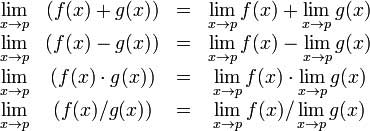Let us consider two functions −

• f(x) = (3x + 5)/(x - 3)
• g(x) = x2 + 1.

Let us calculate the limits of the functions as x tends to 5, of both functions and verify the basic properties of limits using these two functions and MATLAB.

### Example

Create a script file and type the following code into it −

```syms x
f = (3*x + 5)/(x-3);
g = x^2 + 1;
l1 = limit(f, 4)
l2 = limit (g, 4)
lAdd = limit(f + g, 4)
lSub = limit(f - g, 4)
lMult = limit(f*g, 4)
lDiv = limit (f/g, 4)
```

When you run the file, it displays −

```l1 =
17

l2 =
17

34

lSub =
0

lMult =
289

lDiv =
1
```

## Verification of Basic Properties of Limits using Octave

Following is Octave version of the above example using symbolic package, try to execute and compare the result −

```pkg load symbolic
symbols

x = sym("x");
f = (3*x + 5)/(x-3);
g = x^2 + 1;

l1 = subs(f, x, 4)
l2 = subs (g, x, 4)
lAdd = subs (f+g, x, 4)
lSub = subs (f-g, x, 4)
lMult = subs (f*g, x, 4)
lDiv = subs (f/g, x, 4)
```

Octave will execute the above statement and return the following result −

```l1 =
17.0
l2 =
17.0
34.0
lSub =
0.0
lMult =
289.0
lDiv =
1.0
```

## Left and Right Sided Limits

When a function has a discontinuity for some particular value of the variable, the limit does not exist at that point. In other words, limits of a function f(x) has discontinuity at x = a, when the value of limit, as x approaches x from left side, does not equal the value of the limit as x approaches from right side.

This leads to the concept of left-handed and right-handed limits. A left-handed limit is defined as the limit as x -> a, from the left, i.e., x approaches a, for values of x < a. A right-handed limit is defined as the limit as x -> a, from the right, i.e., x approaches a, for values of x > a. When the left-handed limit and right-handed limit are not equal, the limit does not exist.

Let us consider a function −

f(x) = (x - 3)/|x - 3|

We will show that limx->3 f(x) does not exist. MATLAB helps us to establish this fact in two ways −

• By plotting the graph of the function and showing the discontinuity.
• By computing the limits and showing that both are different.

The left-handed and right-handed limits are computed by passing the character strings 'left' and 'right' to the limit command as the last argument.

### Example

Create a script file and type the following code into it −

```f = (x - 3)/abs(x-3);
ezplot(f,[-1,5])
l = limit(f,x,3,'left')
r = limit(f,x,3,'right')
```

When you run the file, MATLAB draws the following plot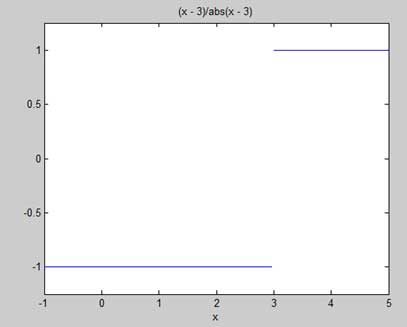After this following output is displayed −

```l =
-1

r =
1
```

# MATLAB - Differential

MATLAB provides the diff command for computing symbolic derivatives. In its simplest form, you pass the function you want to differentiate to diff command as an argument.

For example, let us compute the derivative of the function f(t) = 3t2 + 2t-2

## Example

Create a script file and type the following code into it −

```syms t
f = 3*t^2 + 2*t^(-2);
diff(f)
```

When the above code is compiled and executed, it produces the following result −

```ans =
6*t - 4/t^3
```

Following is Octave equivalent of the above calculation −

```pkg load symbolic
symbols

t = sym("t");
f = 3*t^2 + 2*t^(-2);
differentiate(f,t)
```

Octave executes the code and returns the following result −

```ans =
-(4.0)*t^(-3.0)+(6.0)*t
```

## Verification of Elementary Rules of Differentiation

Let us briefly state various equations or rules for differentiation of functions and verify these rules. For this purpose, we will write f'(x) for a first order derivative and f"(x) for a second order derivative.

Following are the rules for differentiation −

### Rule 1

For any functions f and g and any real numbers a and b are the derivative of the function −

h(x) = af(x) + bg(x) with respect to x is given by −

h'(x) = af'(x) + bg'(x)

### Rule 2

The sum and subtraction rules state that if f and g are two functions, f' and g' are their derivatives respectively, then,

(f + g)' = f' + g'

(f - g)' = f' - g'

### Rule 3

The product rule states that if f and g are two functions, f' and g' are their derivatives respectively, then,

(f.g)' = f'.g + g'.f

### Rule 4

The quotient rule states that if f and g are two functions, f' and g' are their derivatives respectively, then,

(f/g)' = (f'.g - g'.f)/g2

### Rule 5

The polynomial or elementary power rule states that, if y = f(x) = xn, then f' = n. x(n-1)

A direct outcome of this rule is that the derivative of any constant is zero, i.e., if y = k, any constant, then

f' = 0

### Rule 6

The chain rule states that, derivative of the function of a function h(x) = f(g(x)) with respect to x is,

h'(x)= f'(g(x)).g'(x)

### Example

Create a script file and type the following code into it −

```syms x
syms t

f = (x + 2)*(x^2 + 3)
der1 = diff(f)
f = (t^2 + 3)*(sqrt(t) + t^3)
der2 = diff(f)
f = (x^2 - 2*x + 1)*(3*x^3 - 5*x^2 + 2)
der3 = diff(f)
f = (2*x^2 + 3*x)/(x^3 + 1)
der4 = diff(f)
f = (x^2 + 1)^17
der5 = diff(f)
f = (t^3 + 3* t^2 + 5*t -9)^(-6)
der6 = diff(f)
```

When you run the file, MATLAB displays the following result −

```f =
(x^2 + 3)*(x + 2)

der1 =
2*x*(x + 2) + x^2 + 3

f =
(t^(1/2) + t^3)*(t^2 + 3)

der2 =
(t^2 + 3)*(3*t^2 + 1/(2*t^(1/2))) + 2*t*(t^(1/2) + t^3)

f =
(x^2 - 2*x + 1)*(3*x^3 - 5*x^2 + 2)

der3 =
(2*x - 2)*(3*x^3 - 5*x^2 + 2) - (- 9*x^2 + 10*x)*(x^2 - 2*x + 1)

f =
(2*x^2 + 3*x)/(x^3 + 1)

der4 =
(4*x + 3)/(x^3 + 1) - (3*x^2*(2*x^2 + 3*x))/(x^3 + 1)^2

f =
(x^2 + 1)^17

der5 =
34*x*(x^2 + 1)^16

f =
1/(t^3 + 3*t^2 + 5*t - 9)^6

der6 =
-(6*(3*t^2 + 6*t + 5))/(t^3 + 3*t^2 + 5*t - 9)^7
```

Following is Octave equivalent of the above calculation −

```pkg load symbolic
symbols

x = sym("x");
t = sym("t");
f = (x + 2)*(x^2 + 3)
der1 = differentiate(f,x)
f = (t^2 + 3)*(t^(1/2) + t^3)
der2 = differentiate(f,t)
f = (x^2 - 2*x + 1)*(3*x^3 - 5*x^2 + 2)
der3 = differentiate(f,x)
f = (2*x^2 + 3*x)/(x^3 + 1)
der4 = differentiate(f,x)
f = (x^2 + 1)^17
der5 = differentiate(f,x)
f = (t^3 + 3* t^2 + 5*t -9)^(-6)
der6 = differentiate(f,t)
```

Octave executes the code and returns the following result −

```f =

(2.0+x)*(3.0+x^(2.0))
der1 =

3.0+x^(2.0)+(2.0)*(2.0+x)*x
f =

(t^(3.0)+sqrt(t))*(3.0+t^(2.0))
der2 =

(2.0)*(t^(3.0)+sqrt(t))*t+((3.0)*t^(2.0)+(0.5)*t^(-0.5))*(3.0+t^(2.0))
f =

(1.0+x^(2.0)-(2.0)*x)*(2.0-(5.0)*x^(2.0)+(3.0)*x^(3.0))
der3 =

(-2.0+(2.0)*x)*(2.0-(5.0)*x^(2.0)+(3.0)*x^(3.0))+((9.0)*x^(2.0)-(10.0)*x)*(1.0+x^(2.0)-(2.0)*x)
f =

(1.0+x^(3.0))^(-1)*((2.0)*x^(2.0)+(3.0)*x)
der4 =

(1.0+x^(3.0))^(-1)*(3.0+(4.0)*x)-(3.0)*(1.0+x^(3.0))^(-2)*x^(2.0)*((2.0)*x^(2.0)+(3.0)*x)
f =

(1.0+x^(2.0))^(17.0)
der5 =

(34.0)*(1.0+x^(2.0))^(16.0)*x
f =

(-9.0+(3.0)*t^(2.0)+t^(3.0)+(5.0)*t)^(-6.0)
der6 =

-(6.0)*(-9.0+(3.0)*t^(2.0)+t^(3.0)+(5.0)*t)^(-7.0)*(5.0+(3.0)*t^(2.0)+(6.0)*t)
```

## Derivatives of Exponential, Logarithmic and Trigonometric Functions

The following table provides the derivatives of commonly used exponential, logarithmic and trigonometric functions −

Function Derivative
ca.x ca.x.ln c.a (ln is natural logarithm)
ex ex
ln x 1/x
lncx 1/x.ln c
xx xx.(1 + ln x)
sin(x) cos(x)
cos(x) -sin(x)
tan(x) sec2(x), or 1/cos2(x), or 1 + tan2(x)
cot(x) -csc2(x), or -1/sin2(x), or -(1 + cot2(x))
sec(x) sec(x).tan(x)
csc(x) -csc(x).cot(x)

### Example

Create a script file and type the following code into it −

```syms x
y = exp(x)
diff(y)
y = x^9
diff(y)
y = sin(x)
diff(y)
y = tan(x)
diff(y)
y = cos(x)
diff(y)
y = log(x)
diff(y)
y = log10(x)
diff(y)
y = sin(x)^2
diff(y)
y = cos(3*x^2 + 2*x + 1)
diff(y)
y = exp(x)/sin(x)
diff(y)
```

When you run the file, MATLAB displays the following result −

```y =
exp(x)
ans =
exp(x)

y =
x^9
ans =
9*x^8

y =
sin(x)
ans =
cos(x)

y =
tan(x)
ans =
tan(x)^2 + 1

y =
cos(x)
ans =
-sin(x)

y =
log(x)
ans =
1/x

y =
log(x)/log(10)
ans =
1/(x*log(10))

y =
sin(x)^2
ans =
2*cos(x)*sin(x)

y =
cos(3*x^2 + 2*x + 1)
ans =
-sin(3*x^2 + 2*x + 1)*(6*x + 2)

y =
exp(x)/sin(x)
ans =
exp(x)/sin(x) - (exp(x)*cos(x))/sin(x)^2
```

Following is Octave equivalent of the above calculation −

```pkg load symbolic
symbols

x = sym("x");
y = Exp(x)
differentiate(y,x)

y = x^9
differentiate(y,x)

y = Sin(x)
differentiate(y,x)

y = Tan(x)
differentiate(y,x)

y = Cos(x)
differentiate(y,x)

y = Log(x)
differentiate(y,x)

% symbolic packages does not have this support
%y = Log10(x)
%differentiate(y,x)

y = Sin(x)^2
differentiate(y,x)

y = Cos(3*x^2 + 2*x + 1)
differentiate(y,x)

y = Exp(x)/Sin(x)
differentiate(y,x)
```

Octave executes the code and returns the following result −

```y =

exp(x)
ans =

exp(x)
y =

x^(9.0)
ans =

(9.0)*x^(8.0)
y =

sin(x)
ans =

cos(x)
y =

tan(x)
ans =

1+tan(x)^2
y =

cos(x)
ans =

-sin(x)
y =

log(x)
ans =

x^(-1)
y =

sin(x)^(2.0)
ans =

(2.0)*sin(x)*cos(x)
y =

cos(1.0+(2.0)*x+(3.0)*x^(2.0))
ans =

-(2.0+(6.0)*x)*sin(1.0+(2.0)*x+(3.0)*x^(2.0))
y =

sin(x)^(-1)*exp(x)
ans =

sin(x)^(-1)*exp(x)-sin(x)^(-2)*cos(x)*exp(x)
```

## Computing Higher Order Derivatives

To compute higher derivatives of a function f, we use the syntax diff(f,n).

Let us compute the second derivative of the function y = f(x) = x .e-3x

```f = x*exp(-3*x);
diff(f, 2)
```

MATLAB executes the code and returns the following result −

```ans =
9*x*exp(-3*x) - 6*exp(-3*x)
```

Following is Octave equivalent of the above calculation −

```pkg load symbolic
symbols

x = sym("x");
f = x*Exp(-3*x);
differentiate(f, x, 2)
```

Octave executes the code and returns the following result −

```ans =

(9.0)*exp(-(3.0)*x)*x-(6.0)*exp(-(3.0)*x)
```

### Example

In this example, let us solve a problem. Given that a function y = f(x) = 3 sin(x) + 7 cos(5x). We will have to find out whether the equation f" + f = -5cos(2x) holds true.

Create a script file and type the following code into it −

```syms x
y = 3*sin(x)+7*cos(5*x);  % defining the function
lhs = diff(y,2)+y;        %evaluting the lhs of the equation
rhs = -5*cos(2*x);        %rhs of the equation
if(isequal(lhs,rhs))
disp('Yes, the equation holds true');
else
disp('No, the equation does not hold true');
end
disp('Value of LHS is: '), disp(lhs);
```

When you run the file, it displays the following result −

```No, the equation does not hold true
Value of LHS is:
-168*cos(5*x)
```

Following is Octave equivalent of the above calculation −

```pkg load symbolic
symbols

x = sym("x");
y = 3*Sin(x)+7*Cos(5*x);           % defining the function
lhs = differentiate(y, x, 2) + y;  %evaluting the lhs of the equation
rhs = -5*Cos(2*x);                 %rhs of the equation

if(lhs == rhs)
disp('Yes, the equation holds true');
else
disp('No, the equation does not hold true');
end
disp('Value of LHS is: '), disp(lhs);
```

Octave executes the code and returns the following result −

```No, the equation does not hold true
Value of LHS is:
-(168.0)*cos((5.0)*x)
```

## Finding the Maxima and Minima of a Curve

If we are searching for the local maxima and minima for a graph, we are basically looking for the highest or lowest points on the graph of the function at a particular locality, or for a particular range of values of the symbolic variable.

For a function y = f(x) the points on the graph where the graph has zero slope are called stationary points. In other words stationary points are where f'(x) = 0.

To find the stationary points of a function we differentiate, we need to set the derivative equal to zero and solve the equation.

### Example

Let us find the stationary points of the function f(x) = 2x3 + 3x2 − 12x + 17

Take the following steps −

First let us enter the function and plot its graph.

```syms x
y = 2*x^3 + 3*x^2 - 12*x + 17;   % defining the function
ezplot(y)
```

MATLAB executes the code and returns the following plot −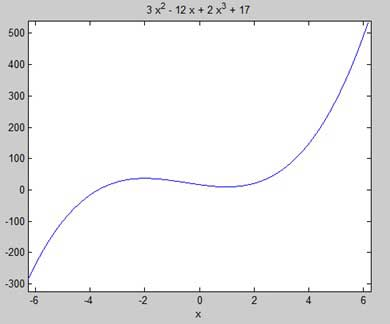Here is Octave equivalent code for the above example −

```pkg load symbolic
symbols

x = sym('x');
y = inline("2*x^3 + 3*x^2 - 12*x + 17");

ezplot(y)
print -deps graph.eps
```

Our aim is to find some local maxima and minima on the graph, so let us find the local maxima and minima for the interval [-2, 2] on the graph.

```syms x
y = 2*x^3 + 3*x^2 - 12*x + 17;   % defining the function
ezplot(y, [-2, 2])
```

MATLAB executes the code and returns the following plot −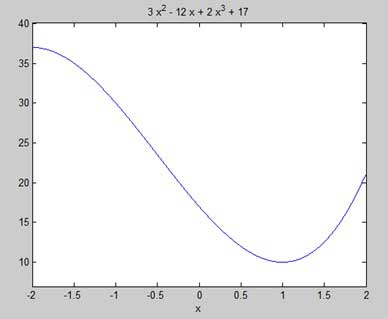Here is Octave equivalent code for the above example −

```pkg load symbolic
symbols

x = sym('x');
y = inline("2*x^3 + 3*x^2 - 12*x + 17");

ezplot(y, [-2, 2])
print -deps graph.eps
```

Next, let us compute the derivative.

```g = diff(y)
```

MATLAB executes the code and returns the following result −

```g =
6*x^2 + 6*x - 12
```

Here is Octave equivalent of the above calculation −

```pkg load symbolic
symbols

x = sym("x");
y = 2*x^3 + 3*x^2 - 12*x + 17;
g = differentiate(y,x)
```

Octave executes the code and returns the following result −

```g =
-12.0+(6.0)*x+(6.0)*x^(2.0)
```

Let us solve the derivative function, g, to get the values where it becomes zero.

```s = solve(g)
```

MATLAB executes the code and returns the following result −

```s =
1
-2
```

Following is Octave equivalent of the above calculation −

```pkg load symbolic
symbols

x = sym("x");
y = 2*x^3 + 3*x^2 - 12*x + 17;
g = differentiate(y,x)
roots([6, 6, -12])
```

Octave executes the code and returns the following result −

```g =

-12.0+(6.0)*x^(2.0)+(6.0)*x
ans =

-2
1
```

This agrees with our plot. So let us evaluate the function f at the critical points x = 1, -2. We can substitute a value in a symbolic function by using the subs command.

```subs(y, 1), subs(y, -2)
```

MATLAB executes the code and returns the following result −

```ans =
10
ans =
37
```

Following is Octave equivalent of the above calculation −

```pkg load symbolic
symbols

x = sym("x");
y = 2*x^3 + 3*x^2 - 12*x + 17;
g = differentiate(y,x)

roots([6, 6, -12])
subs(y, x, 1), subs(y, x, -2)
```

```ans =
10.0
ans =
37.0-4.6734207789940138748E-18*I
```

Therefore, The minimum and maximum values on the function f(x) = 2x3 + 3x2 − 12x + 17, in the interval [-2,2] are 10 and 37.

## Solving Differential Equations

MATLAB provides the dsolve command for solving differential equations symbolically.

The most basic form of the dsolve command for finding the solution to a single equation is

```dsolve('eqn')
```

where eqn is a text string used to enter the equation.

It returns a symbolic solution with a set of arbitrary constants that MATLAB labels C1, C2, and so on.

You can also specify initial and boundary conditions for the problem, as comma-delimited list following the equation as −

```dsolve('eqn','cond1', 'cond2',…)
```

For the purpose of using dsolve command, derivatives are indicated with a D. For example, an equation like f'(t) = -2*f + cost(t) is entered as −

'Df = -2*f + cos(t)'

Higher derivatives are indicated by following D by the order of the derivative.

For example the equation f"(x) + 2f'(x) = 5sin3x should be entered as −

'D2y + 2Dy = 5*sin(3*x)'

Let us take up a simple example of a first order differential equation: y' = 5y.

```s = dsolve('Dy = 5*y')
```

MATLAB executes the code and returns the following result −

```s =
C2*exp(5*t)
```

Let us take up another example of a second order differential equation as: y" - y = 0, y(0) = -1, y'(0) = 2.

```dsolve('D2y - y = 0','y(0) = -1','Dy(0) = 2')
```

MATLAB executes the code and returns the following result −

```ans =
exp(t)/2 - (3*exp(-t))/2
```

# MATLAB - Integration

Integration deals with two essentially different types of problems.

• In the first type, derivative of a function is given and we want to find the function. Therefore, we basically reverse the process of differentiation. This reverse process is known as anti-differentiation, or finding the primitive function, or finding an indefinite integral.

• The second type of problems involve adding up a very large number of very small quantities and then taking a limit as the size of the quantities approaches zero, while the number of terms tend to infinity. This process leads to the definition of the definite integral.

Definite integrals are used for finding area, volume, center of gravity, moment of inertia, work done by a force, and in numerous other applications.

## Finding Indefinite Integral Using MATLAB

By definition, if the derivative of a function f(x) is f'(x), then we say that an indefinite integral of f'(x) with respect to x is f(x). For example, since the derivative (with respect to x) of x2 is 2x, we can say that an indefinite integral of 2x is x2.

In symbols −

f'(x2) = 2x, therefore,

∫ 2xdx = x2.

Indefinite integral is not unique, because derivative of x2 + c, for any value of a constant c, will also be 2x.

This is expressed in symbols as −

∫ 2xdx = x2 + c.

Where, c is called an 'arbitrary constant'.

MATLAB provides an int command for calculating integral of an expression. To derive an expression for the indefinite integral of a function, we write −

```int(f);
```

For example, from our previous example −

```syms x
int(2*x)
```

MATLAB executes the above statement and returns the following result −

```ans =
x^2
```

### Example 1

In this example, let us find the integral of some commonly used expressions. Create a script file and type the following code in it −

```syms x n

int(sym(x^n))
f = 'sin(n*t)'
int(sym(f))
syms a t
int(a*cos(pi*t))
int(a^x)
```

When you run the file, it displays the following result −

```ans =
piecewise([n == -1, log(x)], [n ~= -1, x^(n + 1)/(n + 1)])
f =
sin(n*t)
ans =
-cos(n*t)/n
ans =
(a*sin(pi*t))/pi
ans =
a^x/log(a)
```

### Example 2

Create a script file and type the following code in it −

```syms x n
int(cos(x))
int(exp(x))
int(log(x))
int(x^-1)
int(x^5*cos(5*x))
pretty(int(x^5*cos(5*x)))
int(x^-5)
int(sec(x)^2)
pretty(int(1 - 10*x + 9 * x^2))
int((3 + 5*x -6*x^2 - 7*x^3)/2*x^2)
pretty(int((3 + 5*x -6*x^2 - 7*x^3)/2*x^2))
```

Note that the pretty function returns an expression in a more readable format.

When you run the file, it displays the following result −

```ans =
sin(x)

ans =
exp(x)

ans =
x*(log(x) - 1)

ans =
log(x)

ans =
(24*cos(5*x))/3125 + (24*x*sin(5*x))/625 - (12*x^2*cos(5*x))/125 + (x^4*cos(5*x))/5 - (4*x^3*sin(5*x))/25 + (x^5*sin(5*x))/5
2             4
24 cos(5 x)   24 x sin(5 x)   12 x  cos(5 x)   x  cos(5 x)
----------- + ------------- - -------------- + ------------
3125            625             125              5

3             5

4 x  sin(5 x)   x  sin(5 x)
------------- + -----------
25              5

ans =
-1/(4*x^4)

ans =
tan(x)
2
x (3 x  - 5 x + 1)

ans =
- (7*x^6)/12 - (3*x^5)/5 + (5*x^4)/8 + x^3/2

6      5      4    3
7 x    3 x    5 x    x
- ---- - ---- + ---- + --
12     5      8     2

```

## Finding Definite Integral Using MATLAB

By definition, definite integral is basically the limit of a sum. We use definite integrals to find areas such as the area between a curve and the x-axis and the area between two curves. Definite integrals can also be used in other situations, where the quantity required can be expressed as the limit of a sum.

The int function can be used for definite integration by passing the limits over which you want to calculate the integral.

To calculate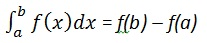we write,

```int(x, a, b)
```

For example, to calculate the value of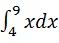we write −

```int(x, 4, 9)
```

MATLAB executes the above statement and returns the following result −

```ans =
65/2
```

Following is Octave equivalent of the above calculation −

```pkg load symbolic
symbols

x = sym("x");
f = x;
c = [1, 0];
integral = polyint(c);

a = polyval(integral, 9) - polyval(integral, 4);
display('Area: '), disp(double(a));
```

Octave executes the code and returns the following result −

```Area:

32.500
```

An alternative solution can be given using quad() function provided by Octave as follows −

```pkg load symbolic
symbols

f = inline("x");
[a, ierror, nfneval] = quad(f, 4, 9);

display('Area: '), disp(double(a));
```

Octave executes the code and returns the following result −

```Area:
32.500
```

### Example 1

Let us calculate the area enclosed between the x-axis, and the curve y = x3−2x+5 and the ordinates x = 1 and x = 2.

The required area is given by −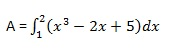Create a script file and type the following code −

```f = x^3 - 2*x +5;
a = int(f, 1, 2)
display('Area: '), disp(double(a));
```

When you run the file, it displays the following result −

```a =
23/4
Area:
5.7500
```

Following is Octave equivalent of the above calculation −

```pkg load symbolic
symbols

x = sym("x");
f = x^3 - 2*x +5;
c = [1, 0, -2, 5];
integral = polyint(c);

a = polyval(integral, 2) - polyval(integral, 1);
display('Area: '), disp(double(a));
```

Octave executes the code and returns the following result −

```Area:

5.7500
```

An alternative solution can be given using quad() function provided by Octave as follows −

```pkg load symbolic
symbols

x = sym("x");
f = inline("x^3 - 2*x +5");

[a, ierror, nfneval] = quad(f, 1, 2);
display('Area: '), disp(double(a));
```

Octave executes the code and returns the following result −

```Area:
5.7500
```

### Example 2

Find the area under the curve: f(x) = x2 cos(x) for −4 ≤ x ≤ 9.

Create a script file and write the following code −

```f = x^2*cos(x);
ezplot(f, [-4,9])
a = int(f, -4, 9)
disp('Area: '), disp(double(a));
```

When you run the file, MATLAB plots the graph −The output is given below −

```a =
8*cos(4) + 18*cos(9) + 14*sin(4) + 79*sin(9)

Area:
0.3326
```

Following is Octave equivalent of the above calculation −

```pkg load symbolic
symbols

x = sym("x");
f = inline("x^2*cos(x)");

ezplot(f, [-4,9])
print -deps graph.eps

[a, ierror, nfneval] = quad(f, -4, 9);
display('Area: '), disp(double(a));
```

# MATLAB - Polynomials

MATLAB represents polynomials as row vectors containing coefficients ordered by descending powers. For example, the equation P(x) = x4 + 7x3 - 5x + 9 could be represented as −

p = [1 7 0 -5 9];

## Evaluating Polynomials

The polyval function is used for evaluating a polynomial at a specified value. For example, to evaluate our previous polynomial p, at x = 4, type −

```p = [1 7 0  -5 9];
polyval(p,4)
```

MATLAB executes the above statements and returns the following result −

```ans = 693
```

MATLAB also provides the polyvalm function for evaluating a matrix polynomial. A matrix polynomial is a polynomial with matrices as variables.

For example, let us create a square matrix X and evaluate the polynomial p, at X −

```p = [1 7 0  -5 9];
X = [1 2 -3 4; 2 -5 6 3; 3 1 0 2; 5 -7 3 8];
polyvalm(p, X)
```

MATLAB executes the above statements and returns the following result −

```ans =
2307       -1769        -939        4499
2314       -2376        -249        4695
2256       -1892        -549        4310
4570       -4532       -1062        9269
```

## Finding the Roots of Polynomials

The roots function calculates the roots of a polynomial. For example, to calculate the roots of our polynomial p, type −

```p = [1 7 0  -5 9];
r = roots(p)
```

MATLAB executes the above statements and returns the following result −

```r =
-6.8661 + 0.0000i
-1.4247 + 0.0000i
0.6454 + 0.7095i
0.6454 - 0.7095i
```

The function poly is an inverse of the roots function and returns to the polynomial coefficients. For example −

```p2 = poly(r)
```

MATLAB executes the above statements and returns the following result −

```p2 =

Columns 1 through 3:

1.00000 + 0.00000i   7.00000 + 0.00000i   0.00000 + 0.00000i

Columns 4 and 5:

-5.00000 - 0.00000i   9.00000 + 0.00000i
```

## Polynomial Curve Fitting

The polyfit function finds the coefficients of a polynomial that fits a set of data in a least-squares sense. If x and y are two vectors containing the x and y data to be fitted to a n-degree polynomial, then we get the polynomial fitting the data by writing −

```p = polyfit(x,y,n)
```

### Example

Create a script file and type the following code −

```x = [1 2 3 4 5 6]; y = [5.5 43.1 128 290.7 498.4 978.67];   %data
p = polyfit(x,y,4)   %get the polynomial

% Compute the values of the polyfit estimate over a finer range,
% and plot the estimate over the real data values for comparison:
x2 = 1:.1:6;
y2 = polyval(p,x2);
plot(x,y,'o',x2,y2)
grid on
```

When you run the file, MATLAB displays the following result −

```p =
4.1056  -47.9607  222.2598 -362.7453  191.1250
```

And plots the following graph −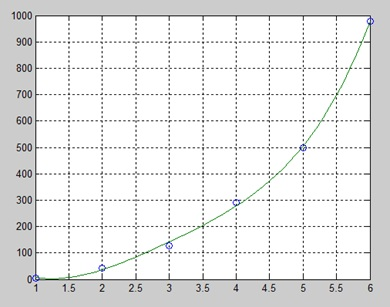# MATLAB - Transforms

MATLAB provides command for working with transforms, such as the Laplace and Fourier transforms. Transforms are used in science and engineering as a tool for simplifying analysis and look at data from another angle.

For example, the Fourier transform allows us to convert a signal represented as a function of time to a function of frequency. Laplace transform allows us to convert a differential equation to an algebraic equation.

MATLAB provides the laplace, fourier and fft commands to work with Laplace, Fourier and Fast Fourier transforms.

## The Laplace Transform

The Laplace transform of a function of time f(t) is given by the following integral −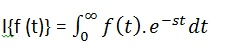Laplace transform is also denoted as transform of f(t) to F(s). You can see this transform or integration process converts f(t), a function of the symbolic variable t, into another function F(s), with another variable s.

Laplace transform turns differential equations into algebraic ones. To compute a Laplace transform of a function f(t), write −

```laplace(f(t))
```

### Example

In this example, we will compute the Laplace transform of some commonly used functions.

Create a script file and type the following code −

```syms s t a b w

laplace(a)
laplace(t^2)
laplace(t^9)
laplace(exp(-b*t))
laplace(sin(w*t))
laplace(cos(w*t))
```

When you run the file, it displays the following result −

```ans =
1/s^2

ans =
2/s^3

ans =
362880/s^10

ans =
1/(b + s)

ans =
w/(s^2 + w^2)

ans =
s/(s^2 + w^2)
```

## The Inverse Laplace Transform

MATLAB allows us to compute the inverse Laplace transform using the command ilaplace.

For example,

```ilaplace(1/s^3)
```

MATLAB will execute the above statement and display the result −

```ans =
t^2/2
```

### Example

Create a script file and type the following code −

```syms s t a b w

ilaplace(1/s^7)
ilaplace(2/(w+s))
ilaplace(s/(s^2+4))
ilaplace(exp(-b*t))
ilaplace(w/(s^2 + w^2))
ilaplace(s/(s^2 + w^2))
```

When you run the file, it displays the following result −

```ans =
t^6/720

ans =
2*exp(-t*w)

ans =
cos(2*t)

ans =
ilaplace(exp(-b*t), t, x)

ans =
sin(t*w)

ans =
cos(t*w)
```

## The Fourier Transforms

Fourier transforms commonly transforms a mathematical function of time, f(t), into a new function, sometimes denoted by or F, whose argument is frequency with units of cycles/s (hertz) or radians per second. The new function is then known as the Fourier transform and/or the frequency spectrum of the function f.

### Example

Create a script file and type the following code in it −

```syms x
f = exp(-2*x^2);     %our function
ezplot(f,[-2,2])     % plot of our function
FT = fourier(f)      % Fourier transform
```

When you run the file, MATLAB plots the following graph −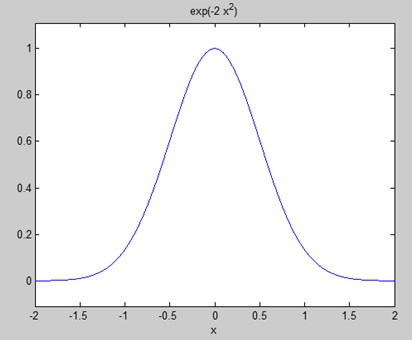The following result is displayed −

```FT =
(2^(1/2)*pi^(1/2)*exp(-w^2/8))/2
```

Plotting the Fourier transform as −

```ezplot(FT)
```

Gives the following graph −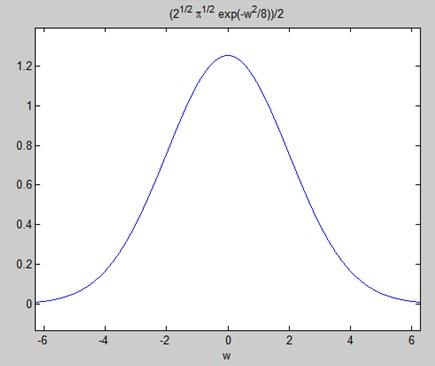## Inverse Fourier Transforms

MATLAB provides the ifourier command for computing the inverse Fourier transform of a function. For example,

```f = ifourier(-2*exp(-abs(w)))
```

MATLAB will execute the above statement and display the result −

```f =
-2/(pi*(x^2 + 1))
```

# MATLAB - GNU Octave Tutorial

GNU Octave is a high-level programming language like MATLAB and it is mostly compatible with MATLAB. It is also used for numerical computations.

Octave has the following common features with MATLAB −

• matrices are fundamental data type
• it has built-in support for complex numbers
• it has built-in math functions and libraries
• it supports user-defined functions

GNU Octave is also freely redistributable software. You may redistribute it and/or modify it under the terms of the GNU General Public License (GPL) as published by the Free Software Foundation.

## MATLAB vs Octave

Most MATLAB programs run in Octave, but some of the Octave programs may not run in MATLAB because, Octave allows some syntax that MATLAB does not.

For example, MATLAB supports single quotes only, but Octave supports both single and double quotes for defining strings. If you are looking for a tutorial on Octave, then kindly go through this tutorial from beginning which covers both MATLAB as well as Octave.

### Compatible Examples

Almost all the examples covered in this tutorial are compatible with MATLAB as well as Octave. Let's try following example in MATLAB and Octave which produces same result without any syntax changes −

This example creates a 3D surface map for the function g = xe-(x2 + y2). Create a script file and type the following code −

```[x,y] = meshgrid(-2:.2:2);
g = x .* exp(-x.^2 - y.^2);
surf(x, y, g)
print -deps graph.eps
```

When you run the file, MATLAB displays the following 3-D map −### Non-compatible Examples

Though all the core functionality of MATLAB is available in Octave, there are some functionality for example, Differential & Integration Calculus, which does not match exactly in both the languages. This tutorial has tried to give both type of examples where they differed in their syntax.

Consider following example where MATLAB and Octave make use of different functions to get the area of a curve: f(x) = x2 cos(x) for −4 ≤ x ≤ 9. Following is MATLAB version of the code −

```f = x^2*cos(x);
ezplot(f, [-4,9])
a = int(f, -4, 9)
disp('Area: '), disp(double(a));
```

When you run the file, MATLAB plots the graph −The following result is displayed

```a =
8*cos(4) + 18*cos(9) + 14*sin(4) + 79*sin(9)

Area:
0.3326
```

But to give area of the same curve in Octave, you will have to make use of symbolic package as follows −

```pkg load symbolic
symbols

x = sym("x");
f = inline("x^2*cos(x)");

ezplot(f, [-4,9])
print -deps graph.eps

[a, ierror, nfneval] = quad(f, -4, 9);
display('Area: '), disp(double(a));
```

# MATLAB - Simulink

Simulink is a simulation and model-based design environment for dynamic and embedded systems, integrated with MATLAB. Simulink, also developed by MathWorks, is a data flow graphical programming language tool for modelling, simulating and analyzing multi-domain dynamic systems. It is basically a graphical block diagramming tool with customizable set of block libraries.

It allows you to incorporate MATLAB algorithms into models as well as export the simulation results into MATLAB for further analysis.

• system-level design
• simulation
• automatic code generation
• testing and verification of embedded systems

There are several other add-on products provided by MathWorks and third-party hardware and software products that are available for use with Simulink.

The following list gives brief description of some of them −

• Stateflow allows developing state machines and flow charts.

• Simulink Coder allows the generation of C source code for real-time implementation of systems automatically.

• xPC Target together with x86-based real-time systems provide an environment to simulate and test Simulink and Stateflow models in real-time on the physical system.

• Embedded Coder supports specific embedded targets.

• HDL Coder allows to automatically generate synthesizable VHDL and Verilog.

• SimEvents provides a library of graphical building blocks for modelling queuing systems.

Simulink is capable of systematic verification and validation of models through modelling style checking, requirements traceability and model coverage analysis.

Simulink Design Verifier allows you to identify design errors and to generate test case scenarios for model checking.

To open Simulink, type in the MATLAB work space −

```simulink
```

Simulink opens with the Library Browser. The Library Browser is used for building simulation models.On the left side window pane, you will find several libraries categorized on the basis of various systems, clicking on each one will display the design blocks on the right window pane.

## Building Models

To create a new model, click the New button on the Library Browser's toolbar. This opens a new untitled model window.A Simulink model is a block diagram.

Model elements are added by selecting the appropriate elements from the Library Browser and dragging them into the Model window.

Alternately, you can copy the model elements and paste them into the model window.

### Examples

Drag and drop items from the Simulink library to make your project.

For the purpose of this example, two blocks will be used for the simulation - A Source (a signal) and a Sink (a scope). A signal generator (the source) generates an analog signal, which will then be graphically visualized by the scope(the sink).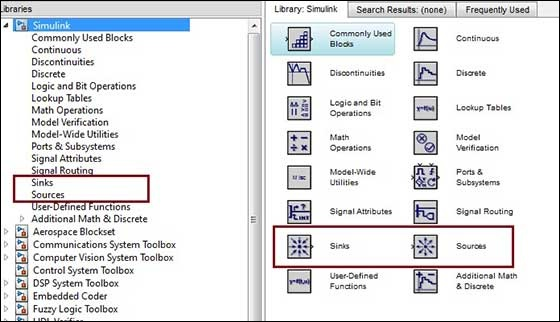Begin by dragging the required blocks from the library to the project window. Then, connect the blocks together which can be done by dragging connectors from connection points on one block to those of another.

Let us drag a 'Sine Wave' block into the model.Select 'Sinks' from the library and drag a 'Scope' block into the model.Drag a signal line from the output of the Sine Wave block to the input of the Scope block.Run the simulation by pressing the 'Run' button, keeping all parameters default (you can change them from the Simulation menu)

You should get the below graph from the scope.